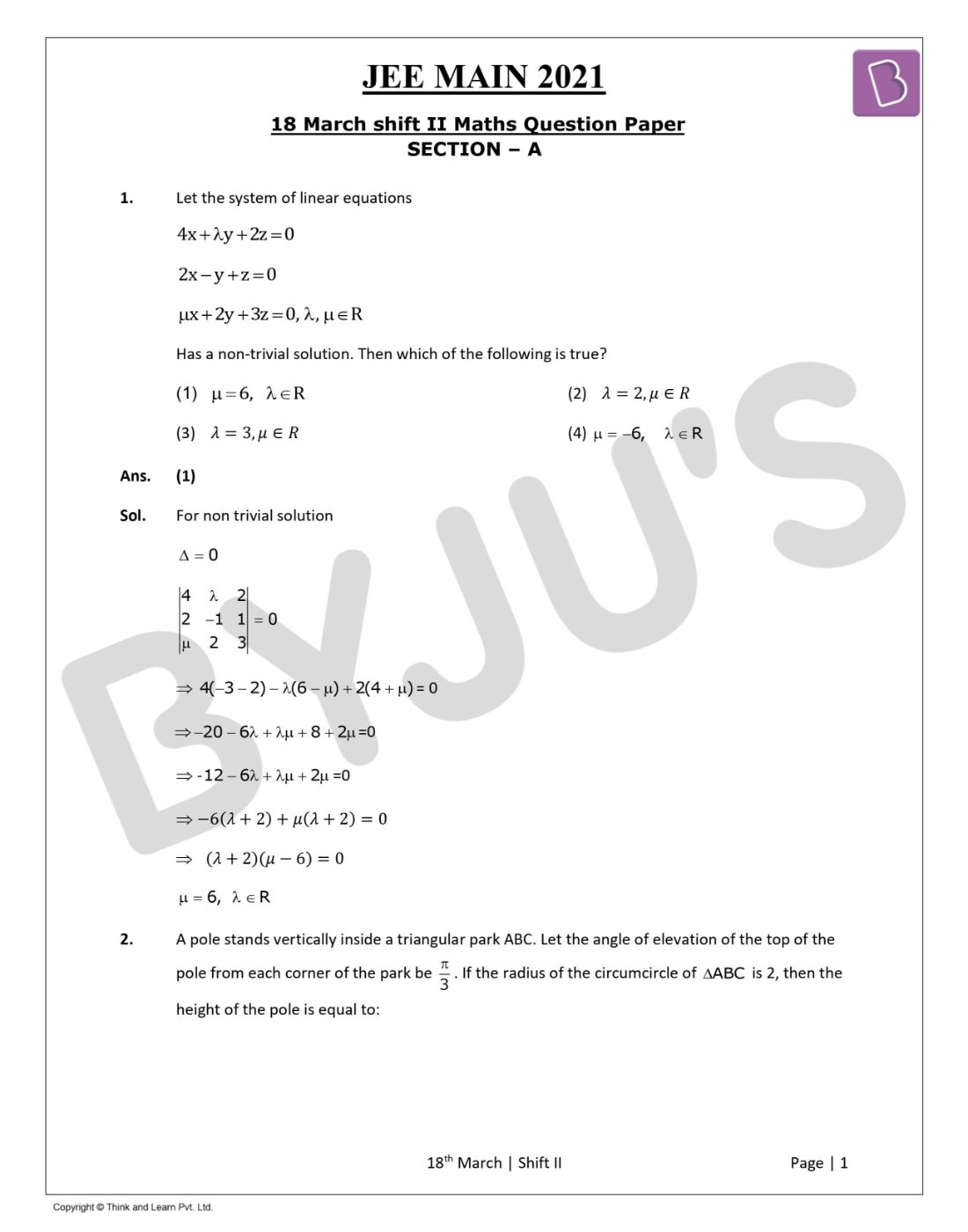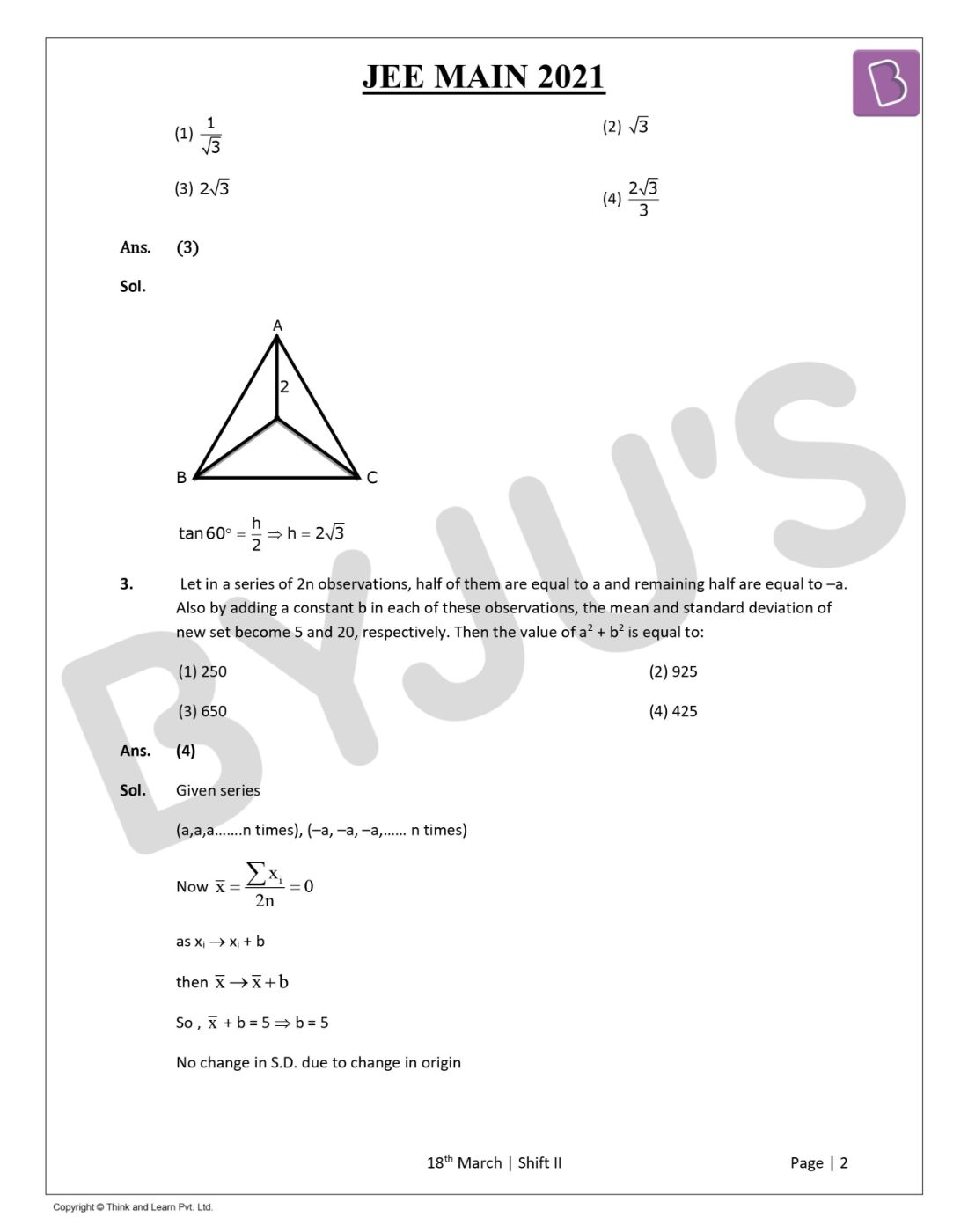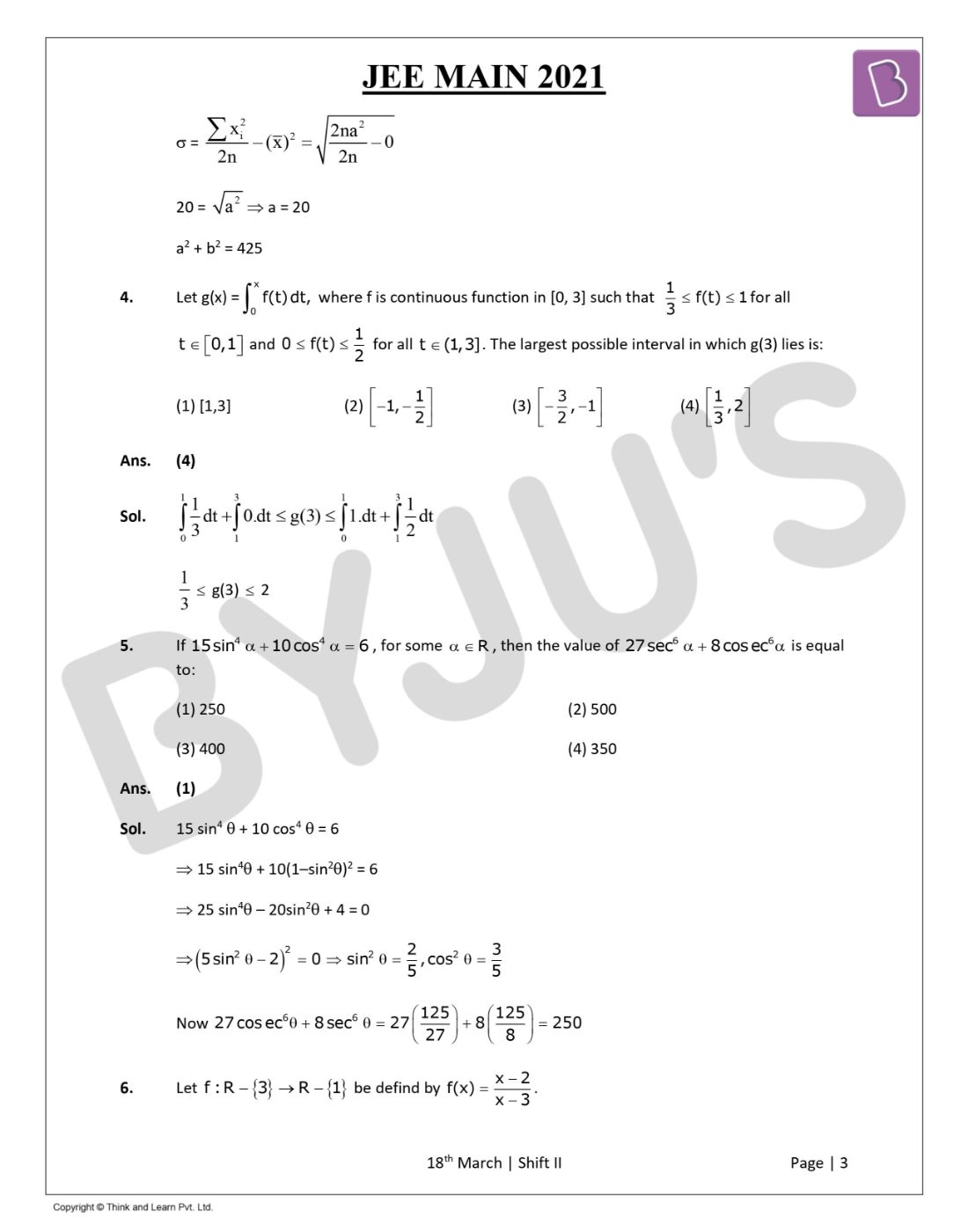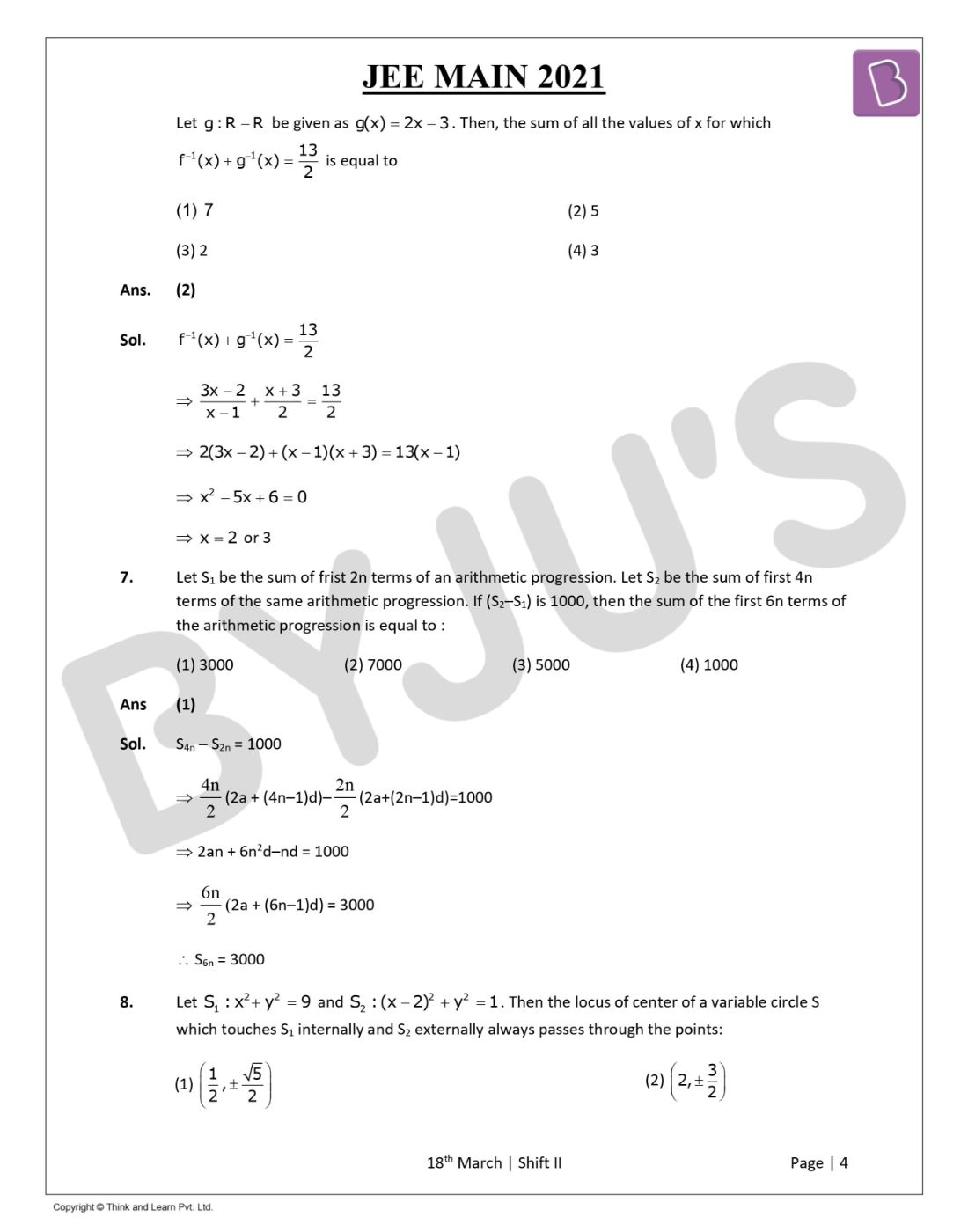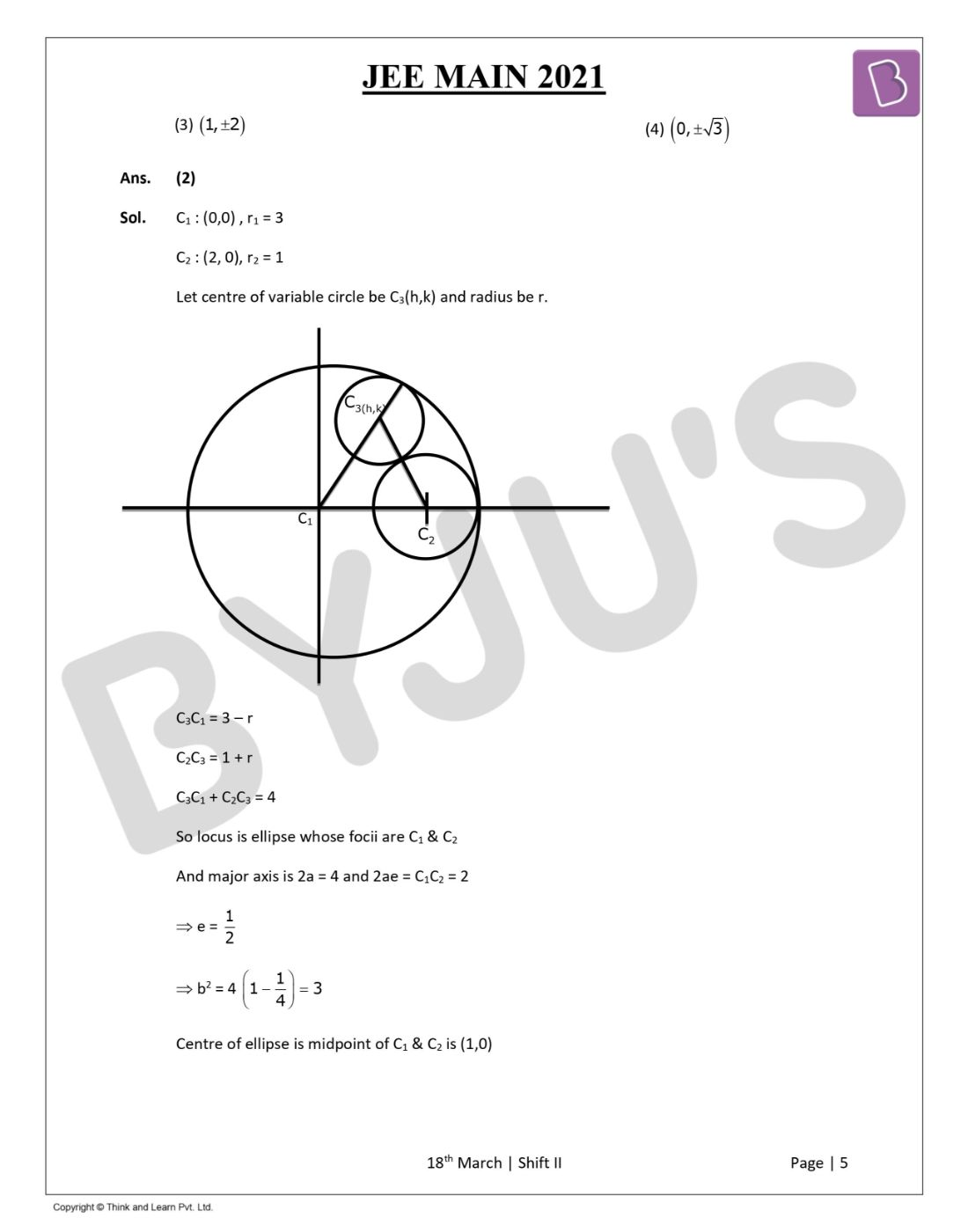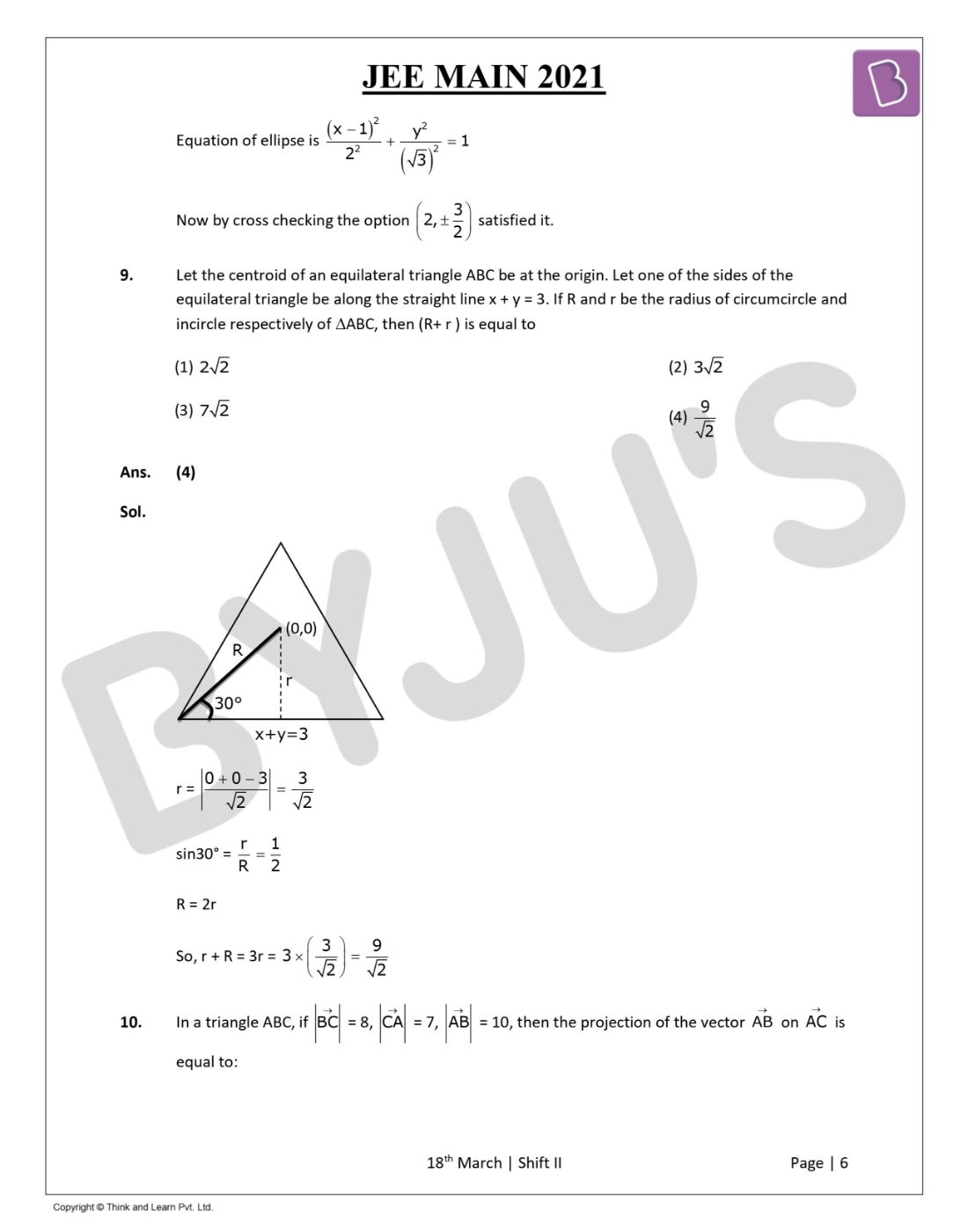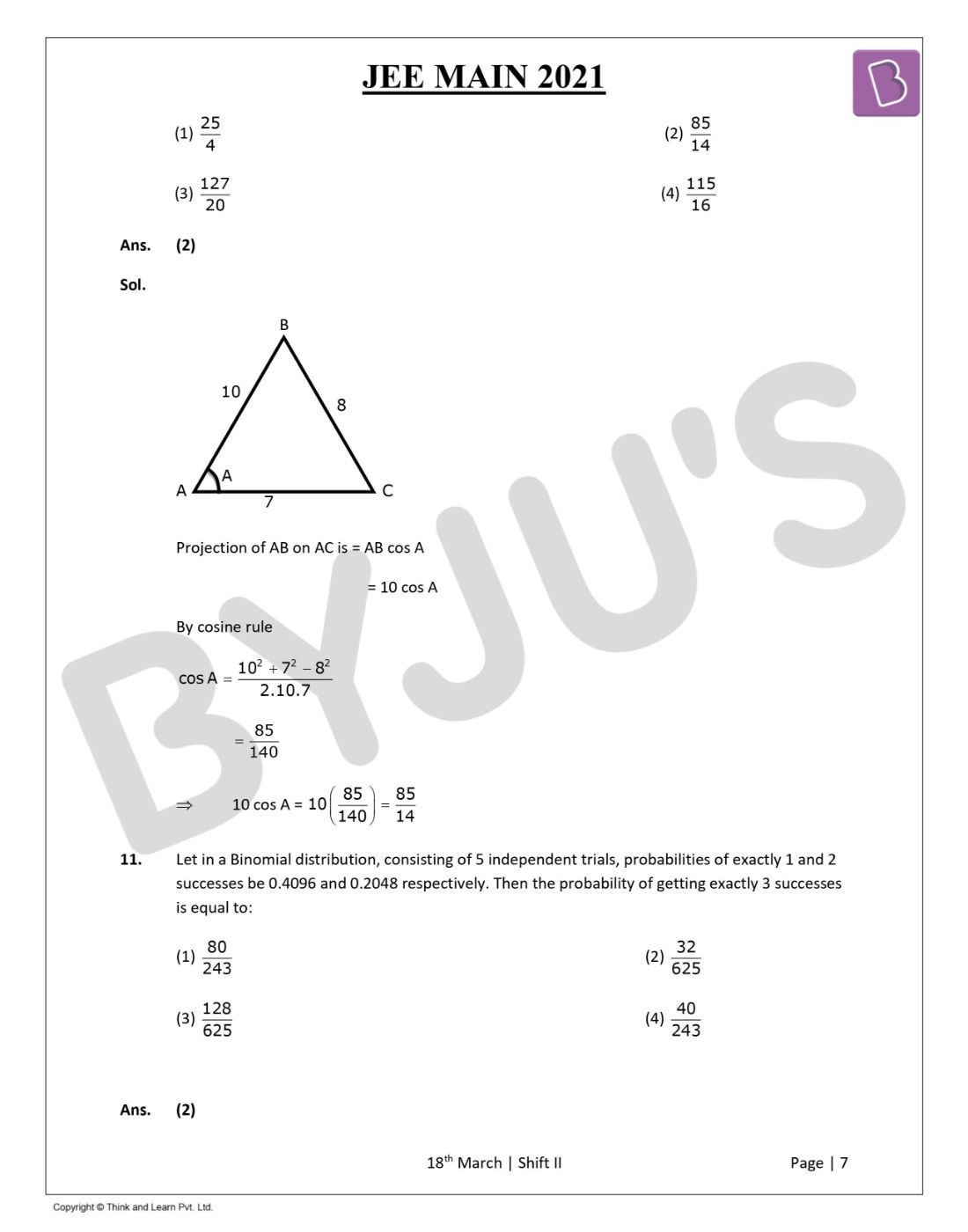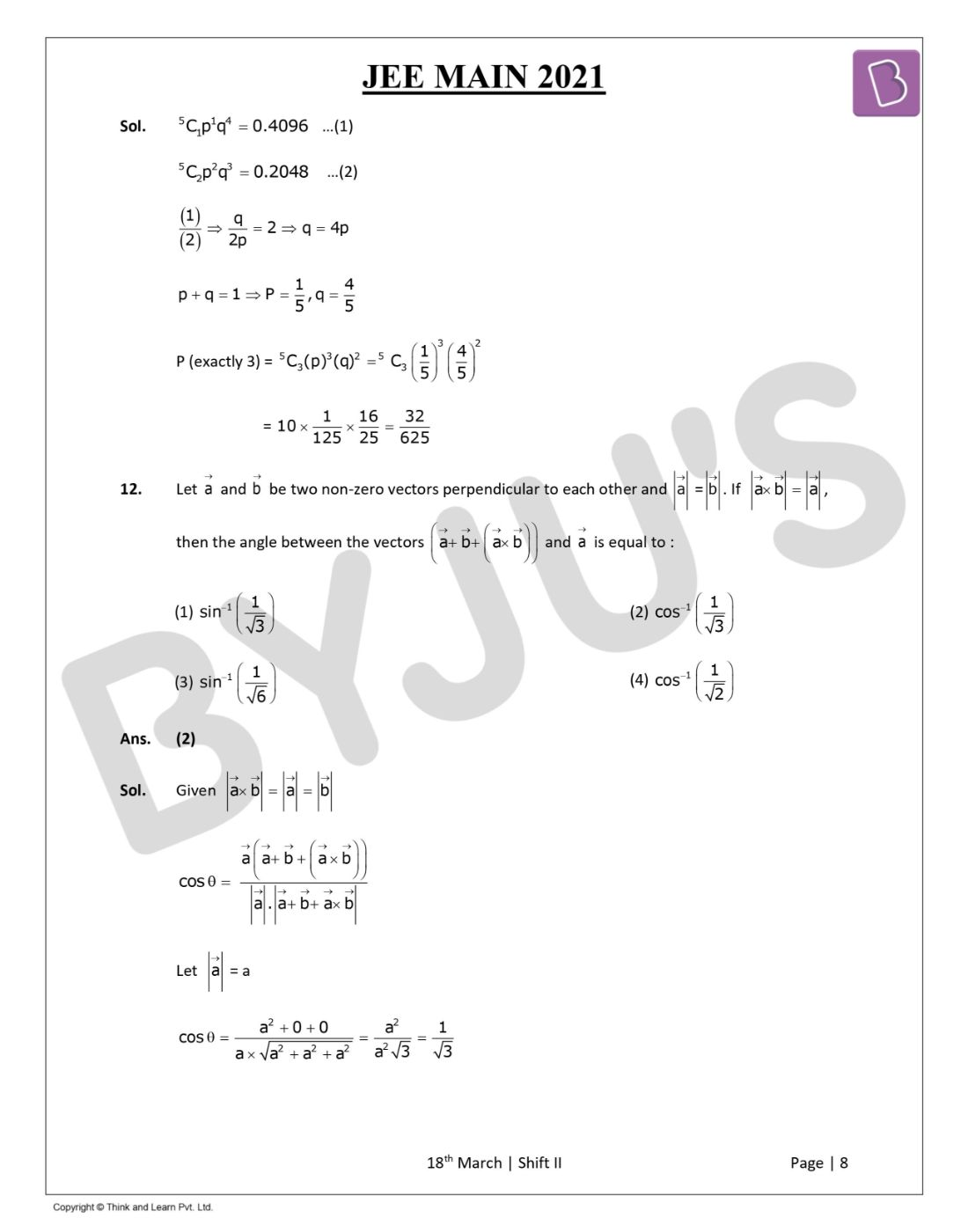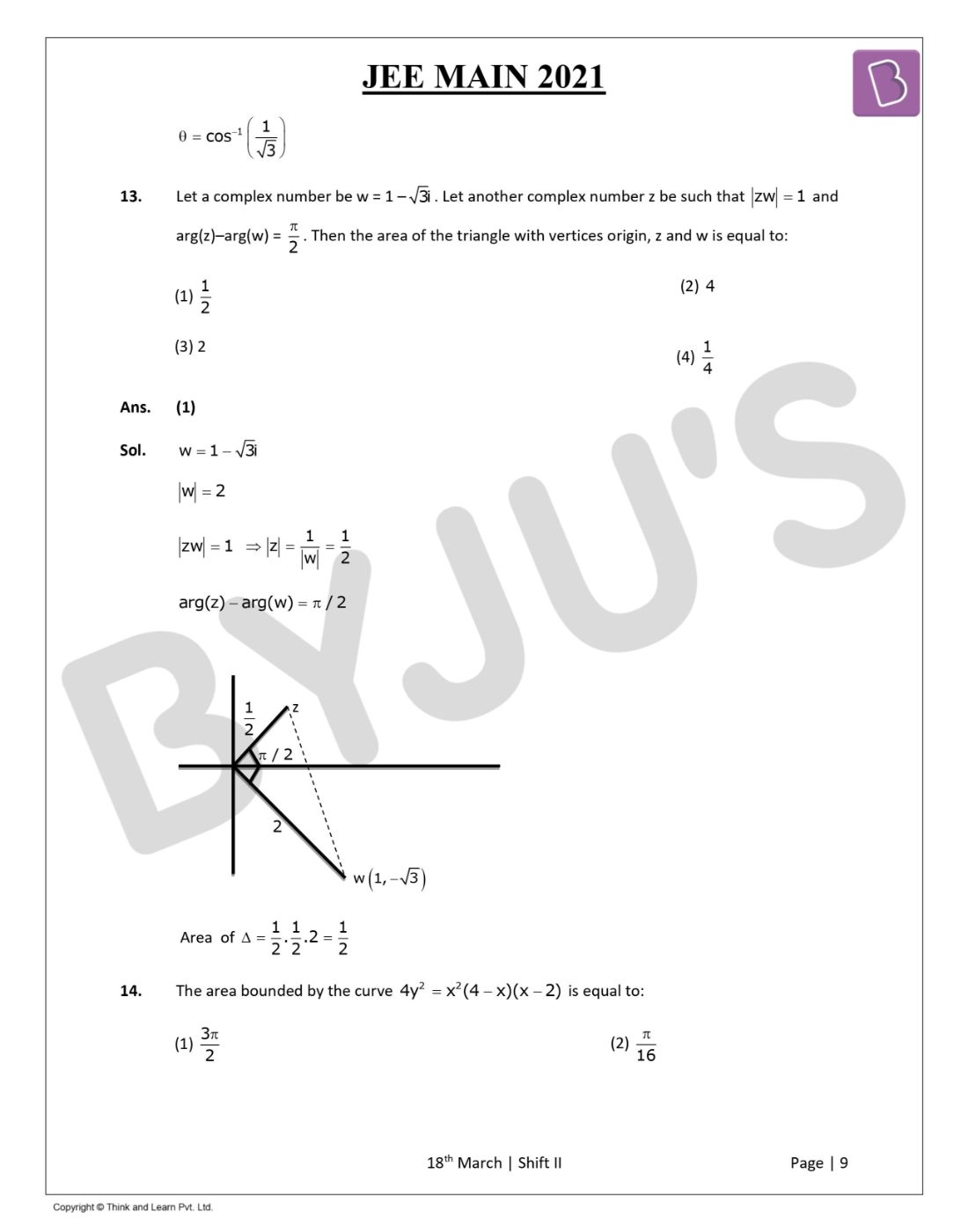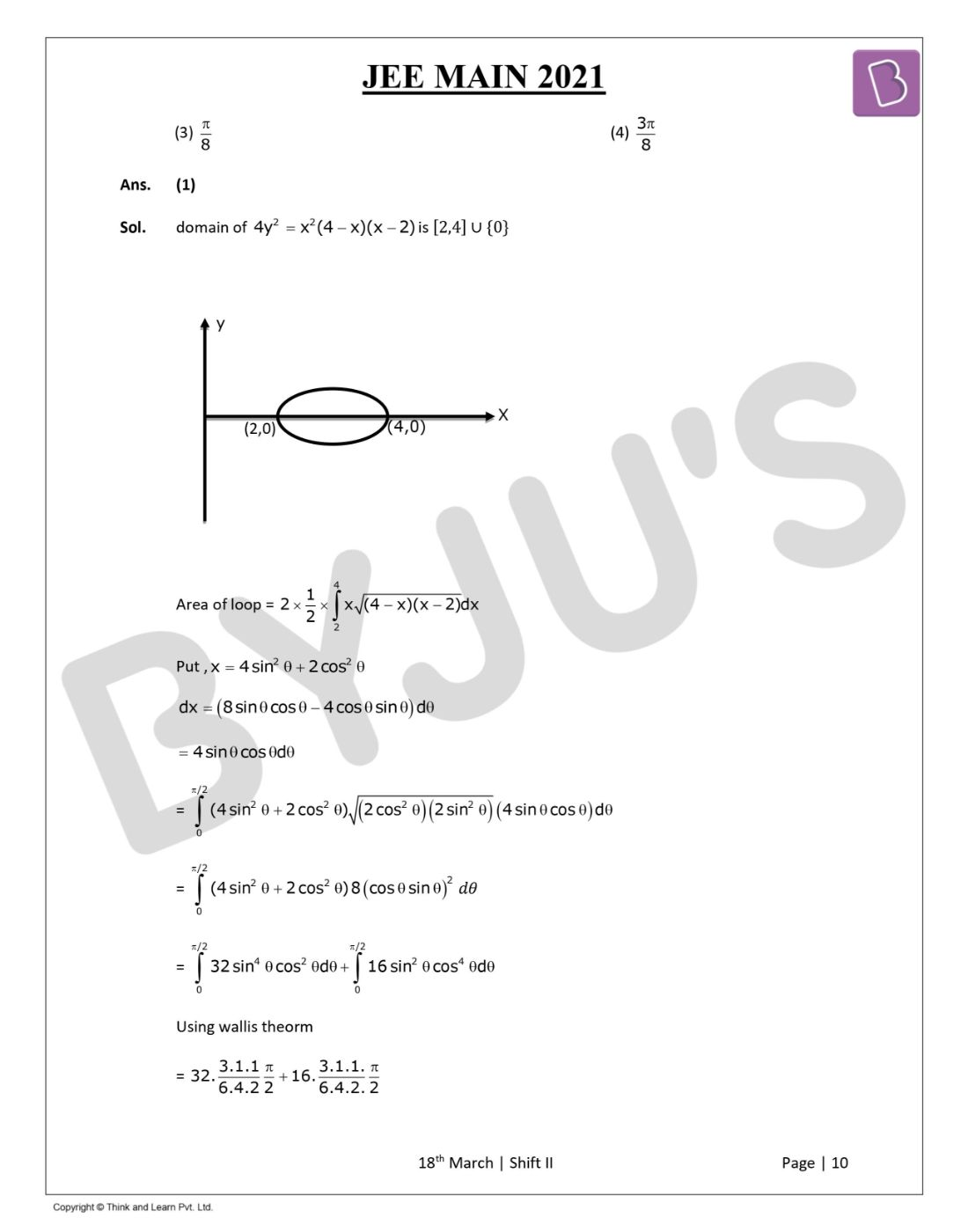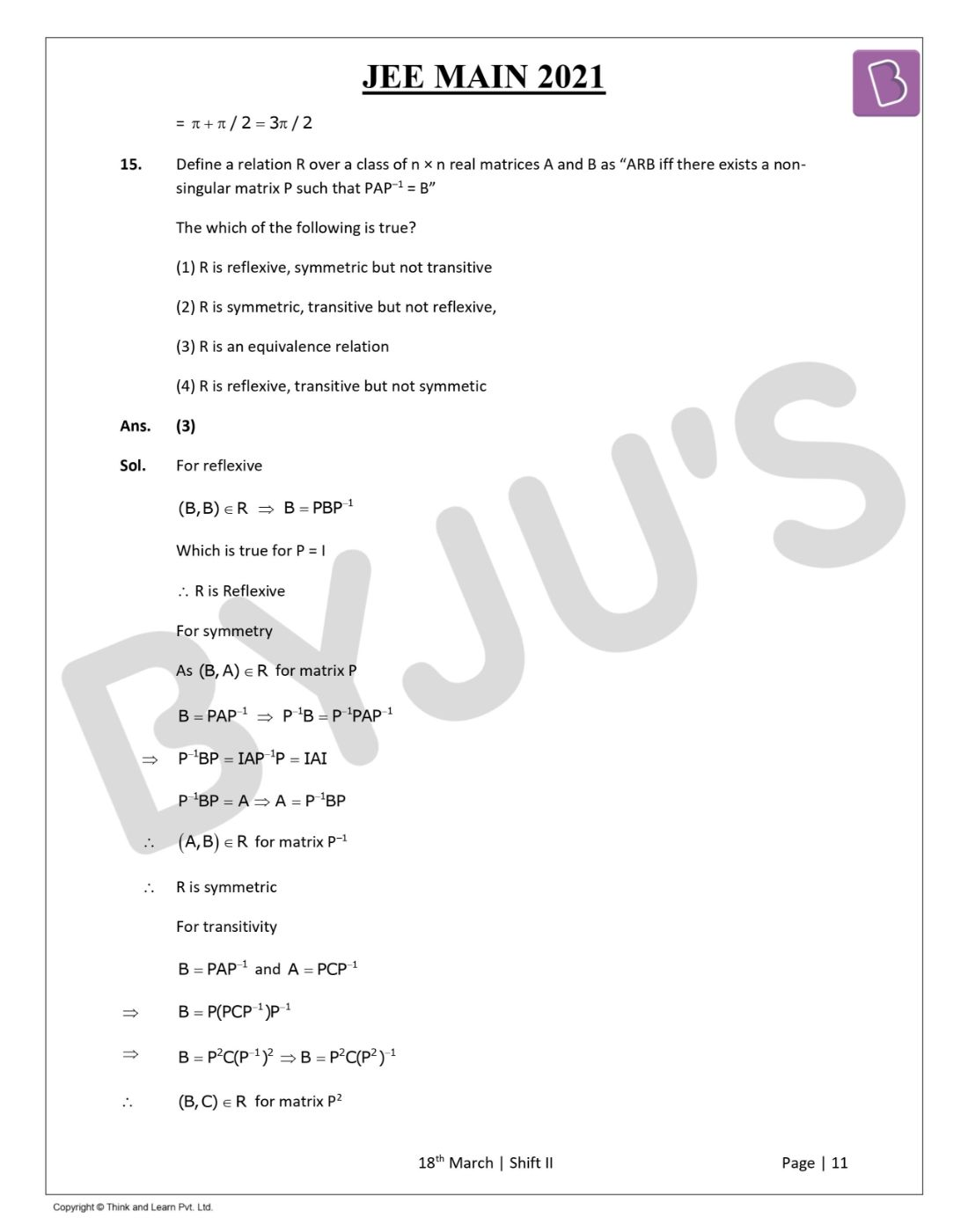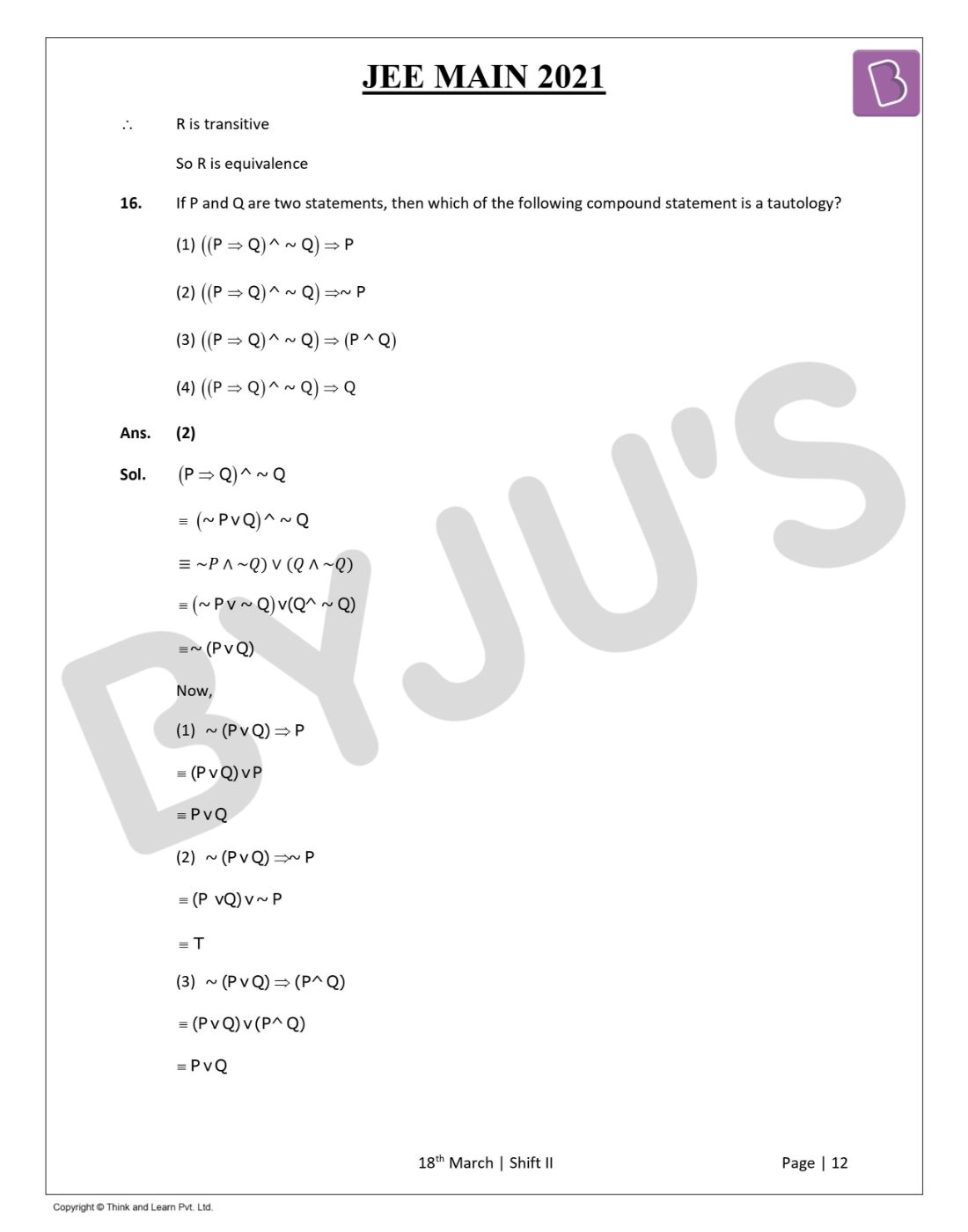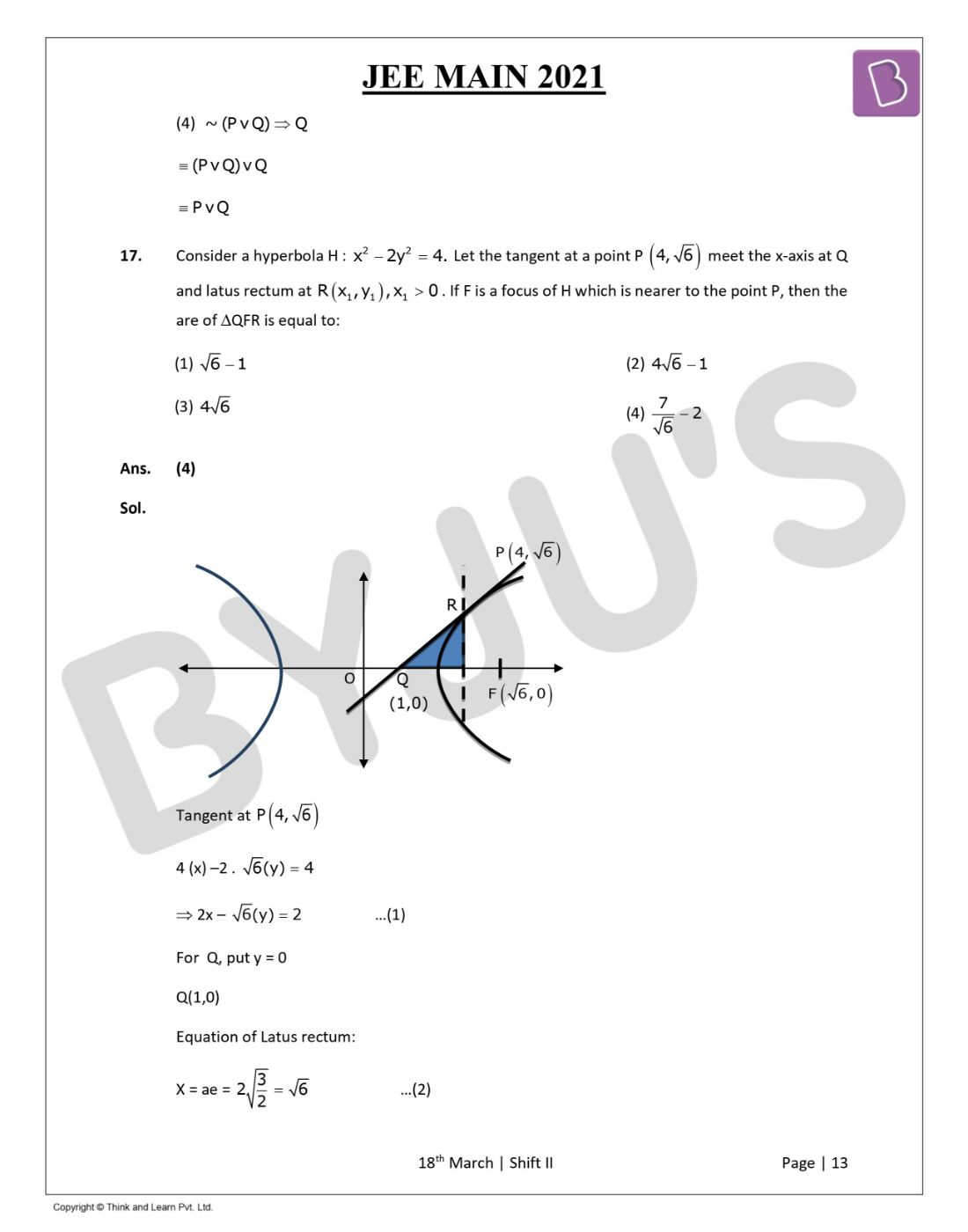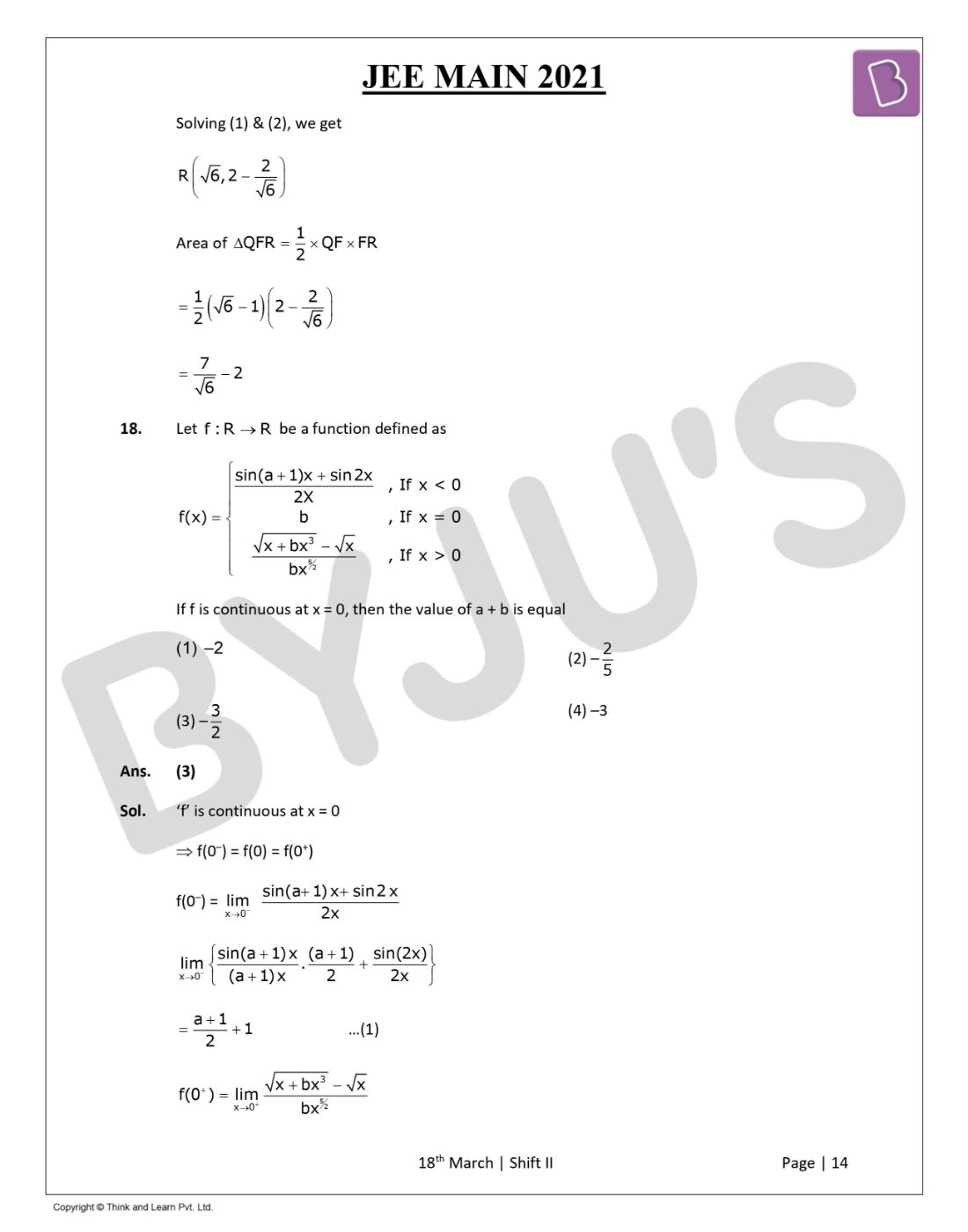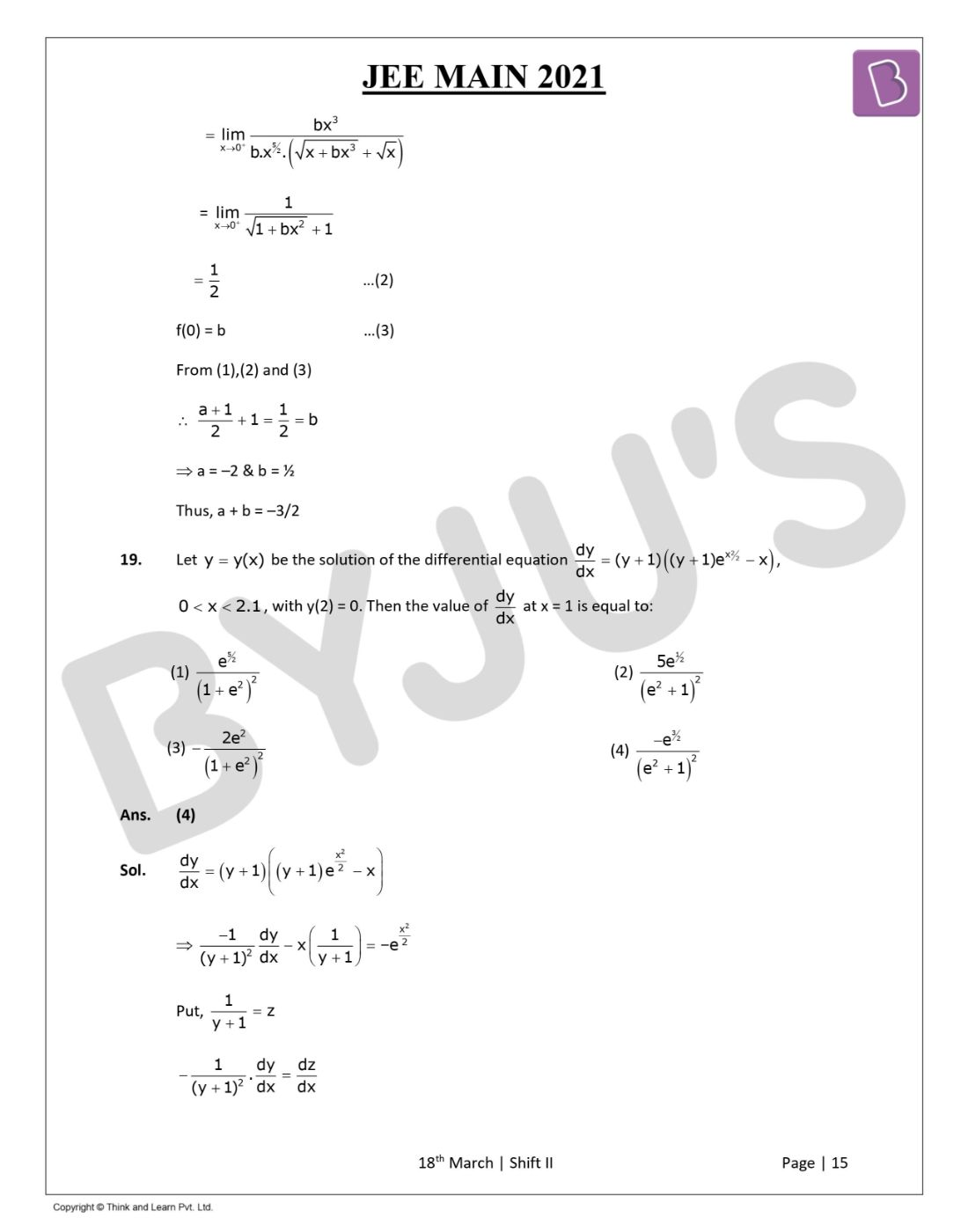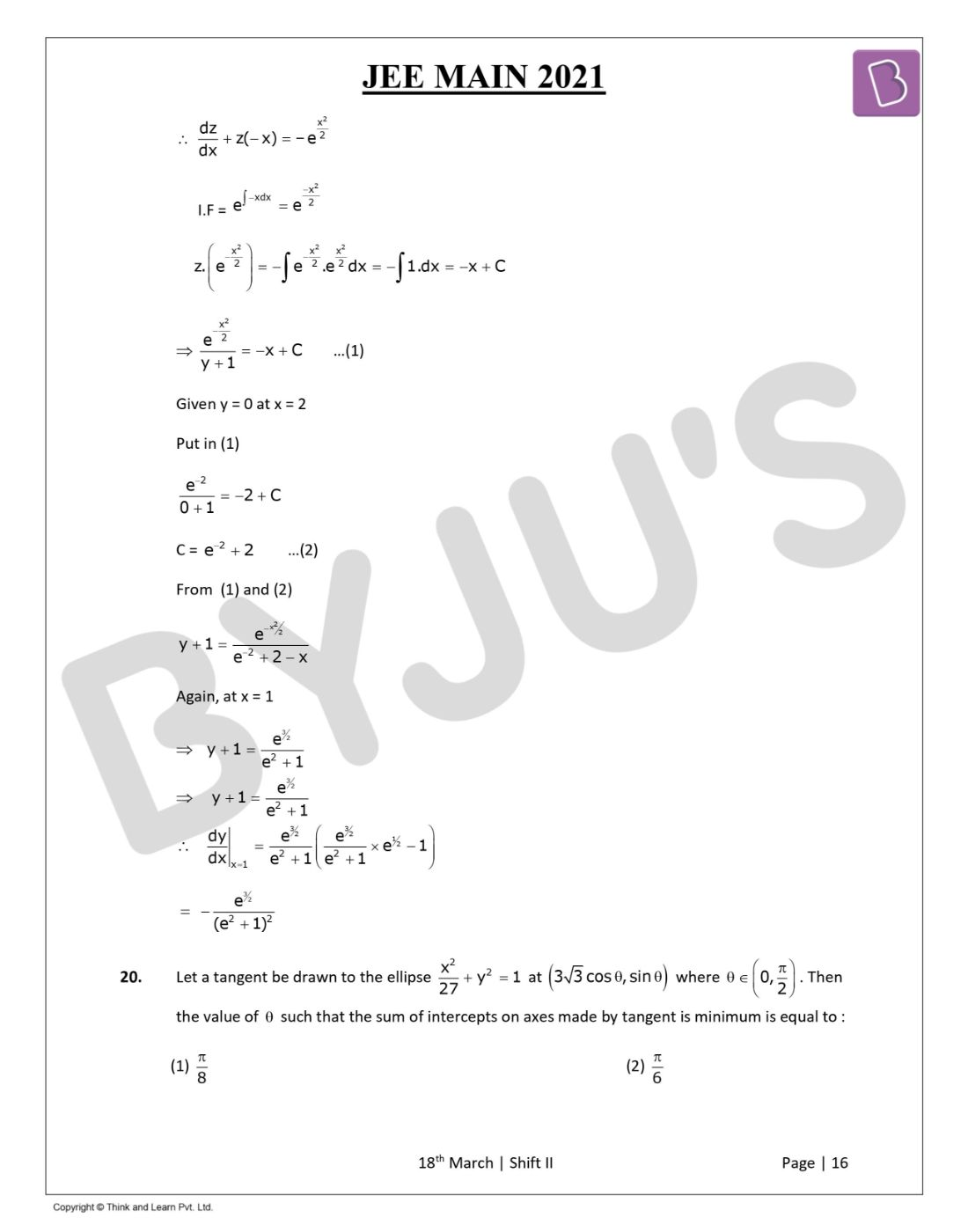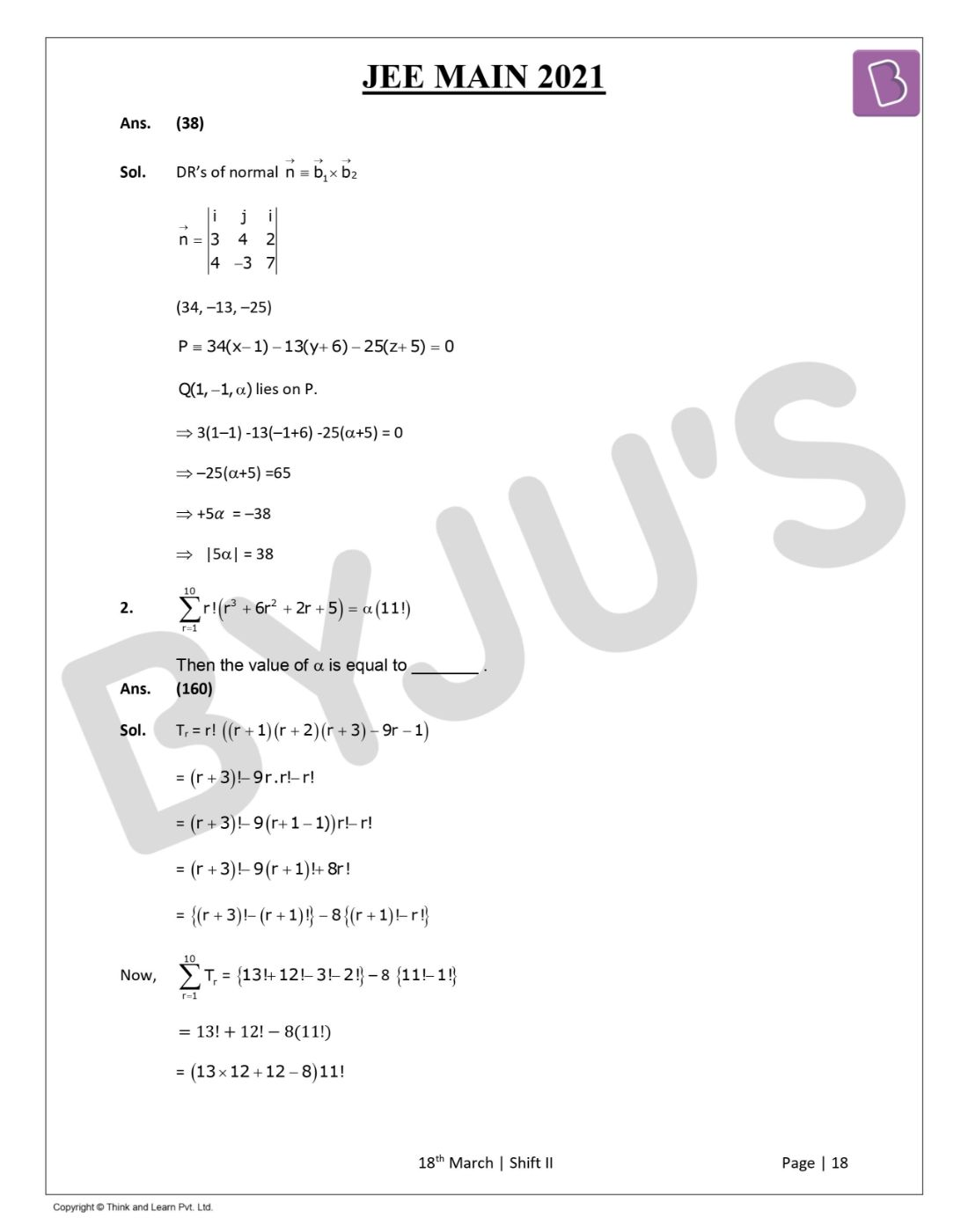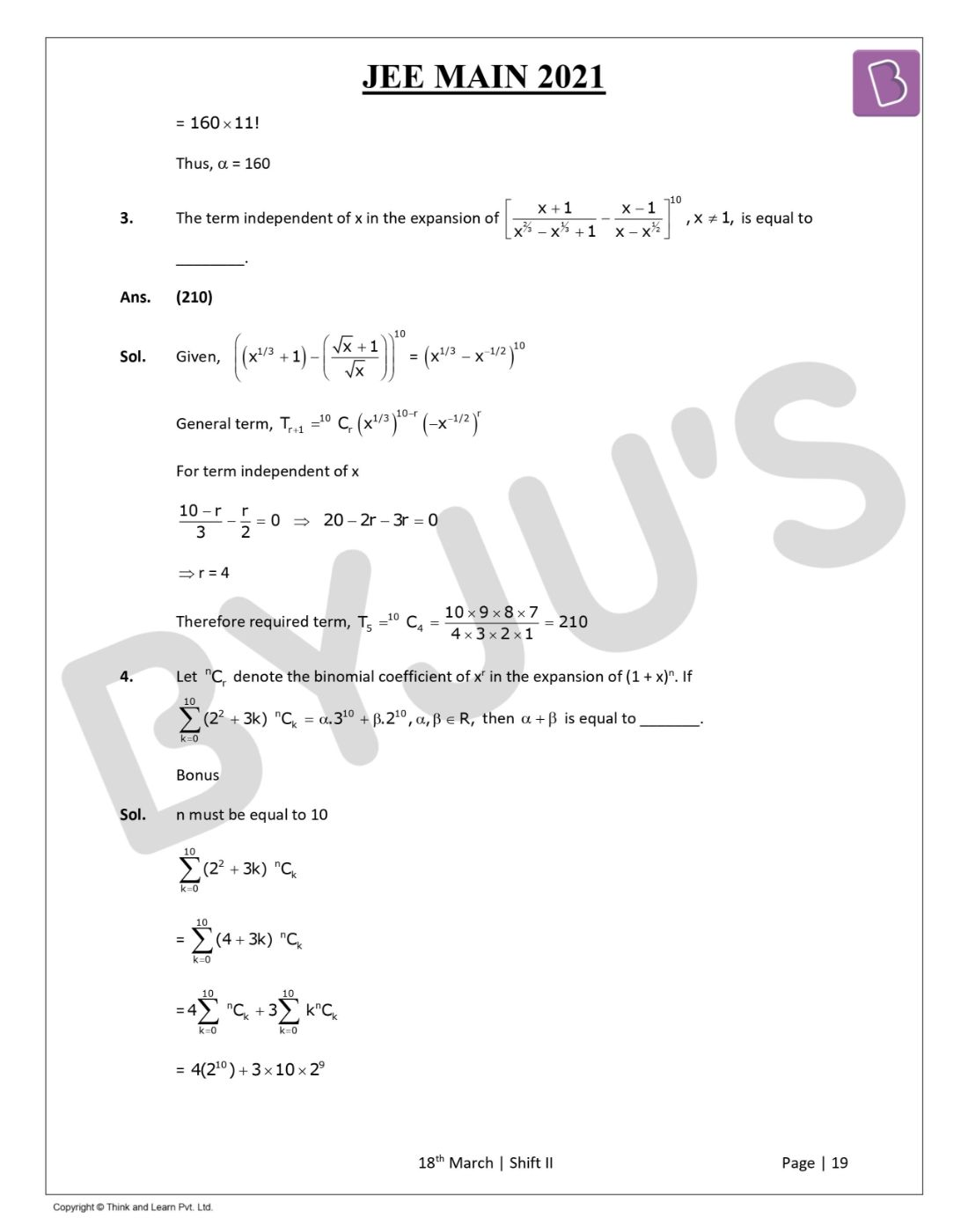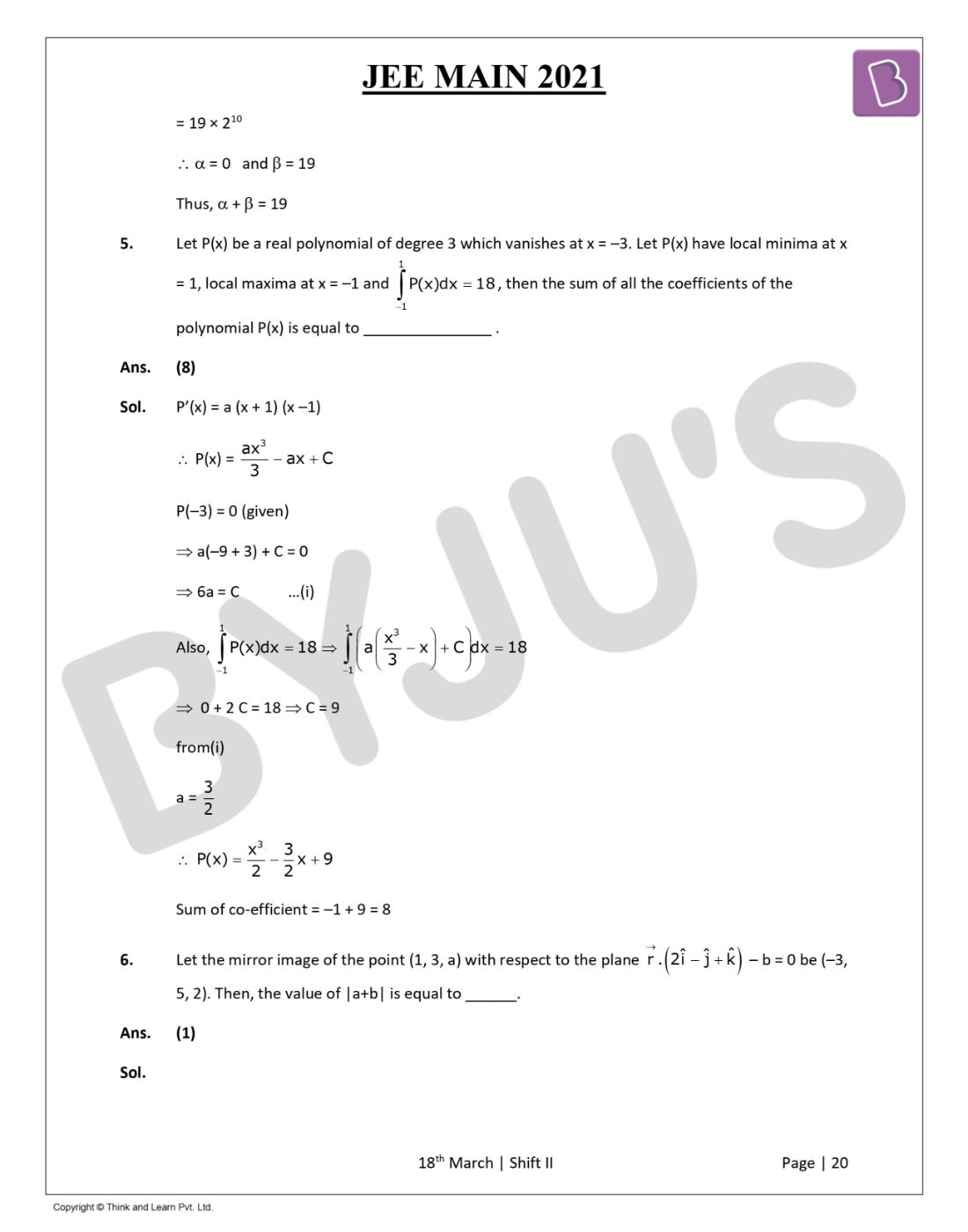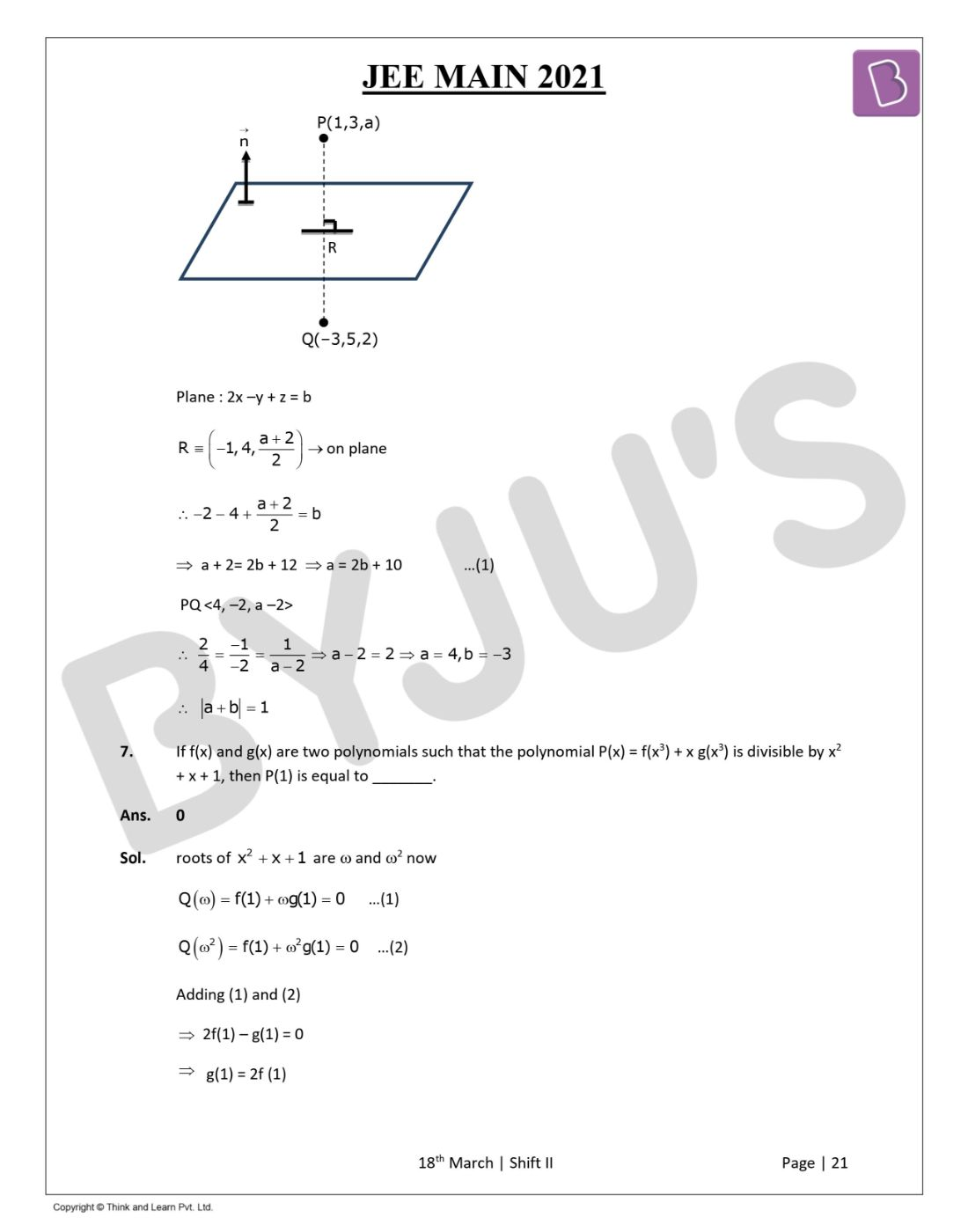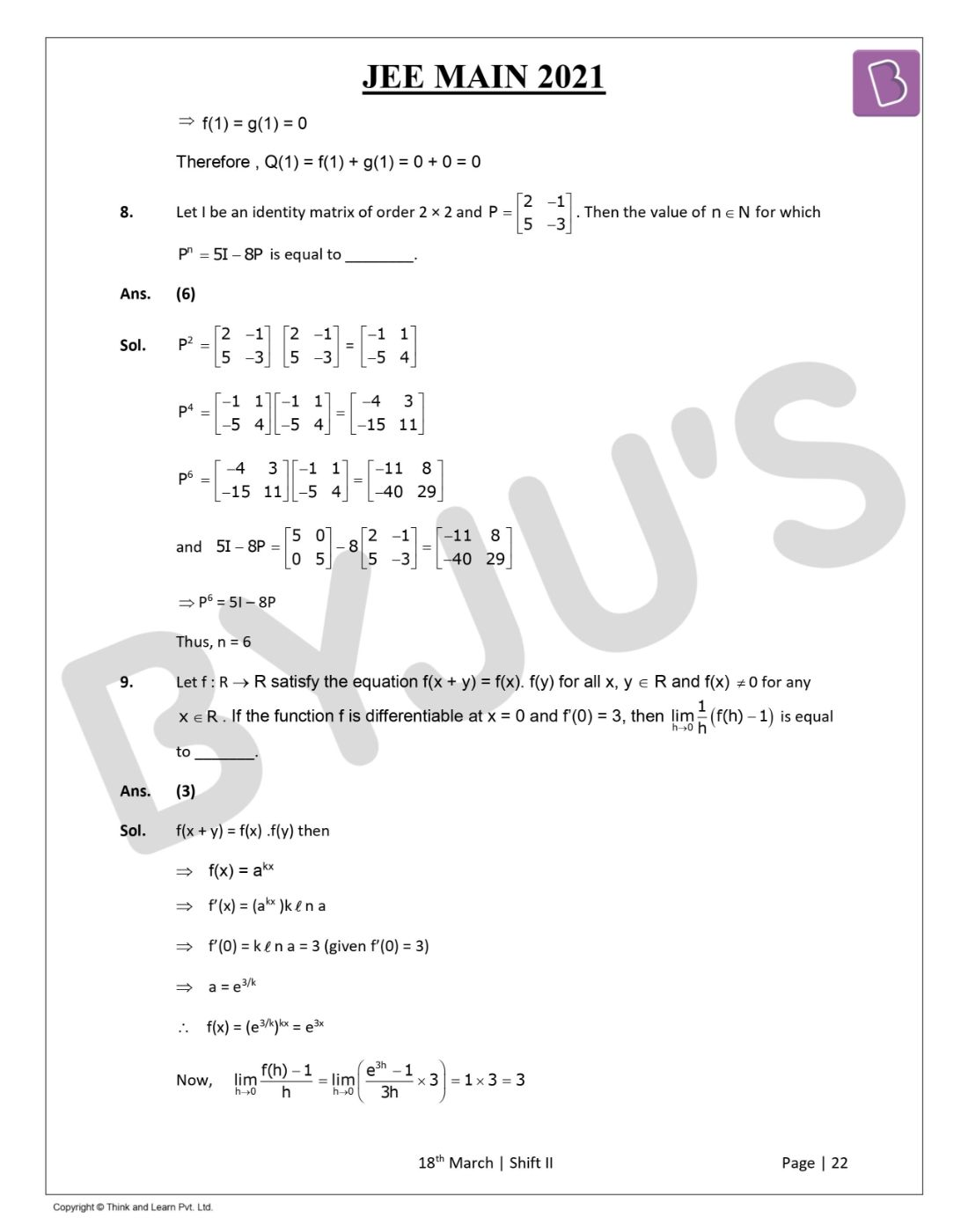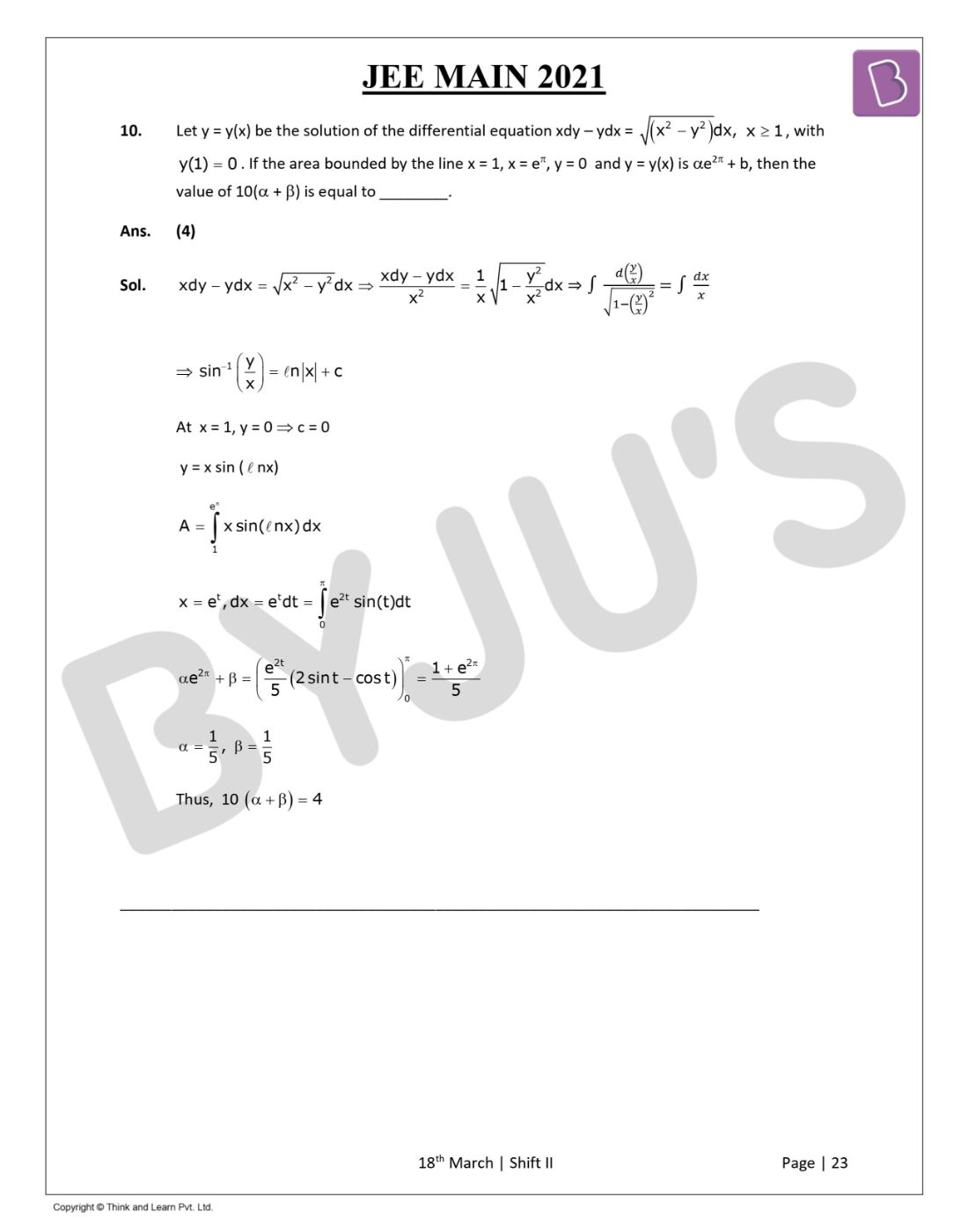Gauge your potential with Aakash BYJU'S JEE Main'23 Mock Test Gauge your potential with Aakash BYJU'S JEE Main'23 Mock Test

# JEE Main 2021 March 18 – Shift 2 Maths Question Paper with Solutions

JEE Main 2021 March 18th Shift 2 Maths question paper are available on this page. The question paper also consists of solutions that have been prepared by experts at BYJU’S to helps students learn the correct answers. Students are advised to go through these solutions so that they can have a better idea about covered concepts as well as the difficulty level and important topics of the JEE Main exam. Students can also download the JEE Main 2021 March 18th Shift 2 Maths question paper PDF for offline use. Additionally, practicing these solutions will definitely help students to improve their problem-solving skills and thereby scoring higher marks.
JEE Main 2021 March 18th Shift 2 Maths Question Paper

Section – A

Question 1: Let the system of linear equations

4x + λy + 2z = 0

2x – y + z = 0

𝝻x + 2y + 3z = 0, λ, 𝝻 ∈ R

Has a non-trivial solution. Then which of the following is true?

a. 𝝻 = 6, λ ∈ R
b. λ = 2, 𝝻 ∈ R
c. λ = 3, 𝝻 ∈ R
d. 𝝻 = – 6, λ ∈ R

For non-trivial solution

Δ = 0

$$\begin{array}{l}\begin{vmatrix} 4 &\lambda &2 \\ 2&-1 &1 \\ \mu&2 &3 \end{vmatrix}=0\end{array}$$

4 (- 3 – 2) – λ (6 – 𝝻) + 2 (4 + 𝝻) = 0

– 20 – 6λ + λ𝝻 + 8 + 2𝝻 = 0

– 12 – 6λ + λ𝝻 + 2𝝻 = 0

– 6 (λ + 2) + 𝝻 (λ + 2) = 0

(λ + 2) (𝝻 – 6) = 0

𝝻 = 6, λ ∈ R

Question 2: A pole stands vertically inside a triangular park ABC. Let the angle of elevation of the top of the pole from each corner of the park be π / 3. If the radius of the circumcircle of △ABC is 2, then the height of the pole is equal to

a. 1 / √3
b. √3
c. 2√3
d. 2√3 / 3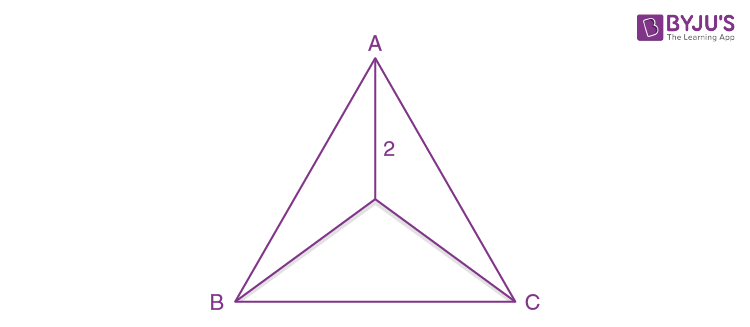tan 60o = h / 2

h = 2√3

Question 3: Let in a series of 2n observations, half of them are equal to a and the remaining half are equal to -a. Also by adding a constant b in each of these observations, the mean and standard deviation of the new set become 5 and 20, respectively. Then the value of a2 + b2 is equal to:

a. 250
b. 925
c. 650
d. 425

Given series

(a,a,a…….n times), (-a, -a, -a,…… n times)

Now

$$\begin{array}{l}\bar{x}=\sum x_{i}/2n=0\end{array}$$

As xi → xi + b, then

$$\begin{array}{l}\bar{x}\rightarrow\bar{x}+b\end{array}$$

So,

$$\begin{array}{l}\bar{x}\end{array}$$
+ b = 5 ⇒ b = 5

No change in S.D. due to change in origin

σ = [∑xi2 / 2n] – (

$$\begin{array}{l}\bar{x}\end{array}$$
)2 = √(2na2 / 2n) – 0

20 = √a2 ⇒ a = 20

a2 + b2 = 425

Question 4: Let g (x) =∫ 0t f (t) dt where f is a continuous function in [0, 3] such that (1 / 3) ≤ f (t) ≤ 1 for all t ∈ [0, 1] and 0 ≤ f (t) for all t ∈ (1, 3]. The largest possible interval in which g (3) lies is:

a. [1, 3]
b. [- 1, – 1 / 2]
c. [- 3 / 2, – 1]
d. [1 / 3, 2]

01 (1 / 3) dt + ∫ 13 0 dt ≤ g (3) ≤ ∫ 01 (1) dt + ∫ 13 (1 / 2) dt

(1 / 3) ≤ g (3) ≤ 2

Question 5: If 15 sin4 θ + 10 cos4 θ = 6, for some θ ∈ R, then the value of 27 sec6 θ + 8 cosec6 θ is equal to:

a. 250
b. 500
c. 400
d. 350

15 sin4 θ + 10 cos4 θ = 6

⇒ 15 sin4 θ + 10 (1 – sin2 θ)2 = 6

⇒ 25 sin4 θ – 20 sin2 θ + 4 = 0

(5 sin2 θ – 2)2 = 0 ⇒ sin2 θ = 2 / 5, cos2 θ = 3 / 5

Now 27 sec6 θ + 8 cosec6 θ = 27 (125 / 27) + 8 (125 / 8) = 250

Question 6: Let f : R – {3} → R – {1] be defined by f (x) = (x – 2) / (x – 3). Let g : R – R be given as g (x) = 2x – 3. Then, the sum of all the values of x for which f-1 (x) + g-1 (x) = 13 / 2 is equal to

a. 7
b. 5
c. 2
d. 3

f-1 (x) + g-1 (x) = 13 / 2

⇒ [3x – 2] / [x – 1] + [x + 3] / 2 = 13 / 2

⇒ 2 (3x – 2) + (x – 1) (x + 3) = 13 (x – 1)

⇒ x2 – 5x + 6 = 0

⇒ x = 2 or 3

Question 7: Let S1 be the sum of the first 2n terms of an arithmetic progression. Let S2 be the sum of the first 4n terms of the same arithmetic progression. If (S2 – S1) is 1000, then the sum of the first 6n terms of the arithmetic progression is equal to :

a. 3000
b. 7000
c. 5000
d. 1000

S4n – S2n = 1000

⇒ (4n / 2) (2a + (4n – 1)d) – (2n / 2) (2a + (2n – 1)d) = 1000

⇒ 2an + 6n2d – nd = 1000

⇒ (6n / 2) (2a + (6n – 1)d) = 3000

∴ S6n = 3000

Question 8: Let S1 = x2 + y2 = 9 and S2 = (x – 2)2 + y2 = 1. Then the locus of the centre of a variable circle S which touches S1 internally and S2 externally always passes through the points:

a. (1 / 2, ± √5 / 2)
b. (2, ± 3 / 2)
c. (1, ± 2)
d. (0, ± √3)

C1 : (0,0) , r1 = 3

C2 : (2, 0), r2 = 1

Let the centre of the variable circle be C3(h, k) and the radius is r.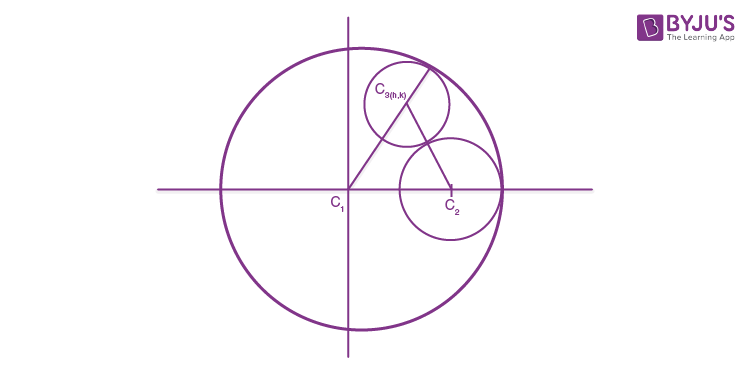C3C1 = 3 – r

C2C3 = 1 + r

C3C1 + C2C3 = 4

So locus is ellipse whose focii are C1 & C2

And major axis is 2a = 4 and 2ae = C1C2 = 2

⇒ e = 1 / 2

⇒ b2 = 4 [1 – (1 / 4)] = 3

The centre of the ellipse is the midpoint of C1 & C2 is (1, 0).

The equation of the ellipse is [x – 1]2 / 22 + [y2] / [√3]2 = 1.

Now by cross-checking the option (2, ± 3 / 2) satisfied it.

Question 9: Let the centroid of an equilateral triangle ABC be at the origin. Let one of the sides of the equilateral triangle be along the straight line x + y = 3. If R and r be the radius of circumcircle and incircle respectively of ΔABC, then (R + r) is equal to

a. 2√2
b. 3√2
c. 7√2
d. 9 / √2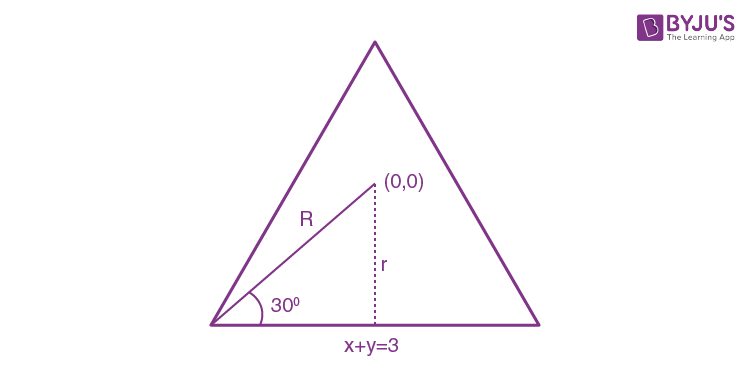r = |(0 + 0 – 3) / √2| = 3 / √2

sin 30° = r / R = 1 / 2

R = 2r

So, r + R = 3r = 3 * (3 / √2) = 9 / √2

Question 10: In a triangle ABC, if vector BC = 8, CA = 7, AB = 10, then the projection of the vector AB on AC is equal to:

a. 25 / 4
b. 85 / 14
c. 127 / 20
d. 115 / 16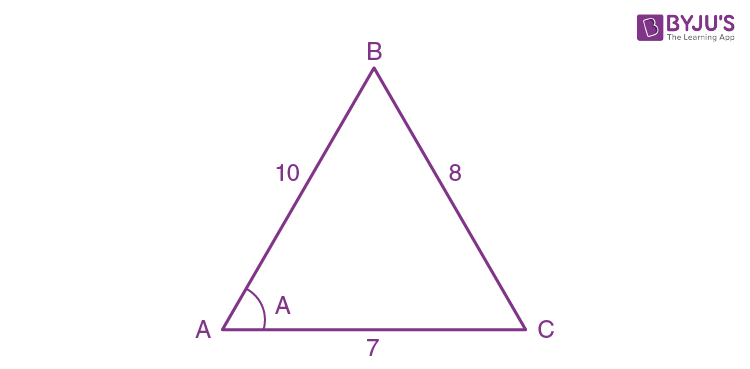Projection of AB on AC is = AB cos A = 10 cos A

By cosine rule

cos A = [102 + 72 – 82] / [2 . 10 . 7]

= 85 / 140

10 cos A = 10 (85 / 140) = 85 / 14

Question 11: Let in a Binomial distribution, consisting of 5 independent trials, probabilities of exactly 1 and 2 successes be 0.4096 and 0.2048 respectively. Then the probability of getting exactly 3 successes is equal to:

a. 80 / 243
b. 32 / 625
c. 128 / 625
d. 40 / 243

5C1 p1 q4 = 0.4096 — (1)

5C2 p2 q3 = 0.2048 — (2)

(1) / (2) ⇒ q / 2p = 2 ⇒ q = 4p

p + q = 1 ⇒ p = 1 / 5 and q = 4 / 5

P (exactly 3) = 5C3 (p)3 (q)2 = 5C3 (1 / 5)3 (4 / 5)2

= (10) * (1 / 125) * (16 / 25) = 32 / 625

Question 12: Let a and b be two non-zero vectors perpendicular to each other and |a| = |b|. If |a x b| = |a|, then the angle between the vectors (a + b + (a x b)) and a is equal to:

a. sin-1 (1 / √3)
b. cos-1 (1 / √3)
c. sin-1 (1 / √6)
d. cos-1 (1 / √2)

$$\begin{array}{l}\\|\vec{a}\times\vec{b}|=|\vec{a}|=|\vec{b}|\\ cos\Theta =\frac{\vec{a}\times(\vec{a}+\vec{b}+(\vec{a}\times\vec{b}))}{|\vec{a}|.|\vec{a}+\vec{b}+\vec{a}\times\vec{b}|}\\ |\vec{a}|=a\\ cos\Theta =\frac{a^{2}+0+0}{a\times\sqrt{a^{2}+a^{2}+a^{2}}}\\ =\frac{a^{2}}{a^{2}\sqrt{3}}\\ =\frac{1}{\sqrt{3}}\\ \Theta =cos^{-1}(\frac{1}{\sqrt{3}})\end{array}$$

Question 13: Let a complex number be w = 1 – √3i. Let another complex number z be such that |zw| = 1 and arg (z) – arg (w) = π / 2. Then the area of the triangle with vertices origin, z and w is equal to:

a. 1 / 2
b. 4
c. 2
d. 1 / 4

w = 1 – √3i

|w| = 2

|zw| = 1 ⇒ |z| = 1 / |w| = 1 / 2

arg (z) – arg (w) = π / 2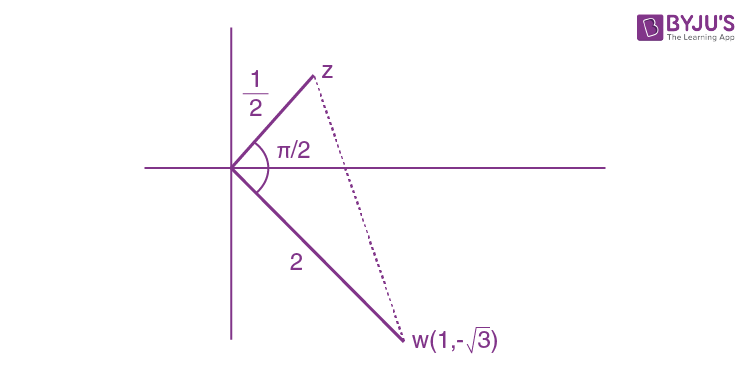Area of triangle = (1 / 2) * (1 / 2) * 2 = 1 / 2

Question 14: The area bounded by the curve 4y2 = x2 (4 – x) (x – 2) is equal to:

a. 3π / 2
b. π / 16
c. π / 8
d. 3π / 8

The domain of 4y2 = x2 (4 – x) (x – 2) is [2, 4] ⋃ {0}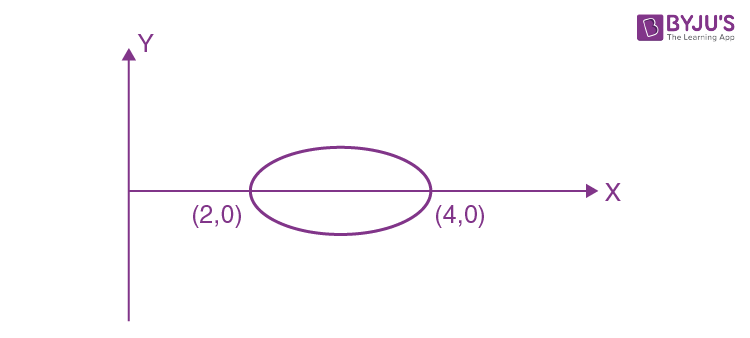Area of loop = 2 * (1 / 2) * ∫24 x √(4 – x) (x – 2) dx

Put x = 4 sin2 θ + 2 cos2 θ

dx = (8 sin θ cos θ – 4 cos θ sin θ) dθ

= 4 sin θ cos θ dθ

= ∫0π/2 (4 sin2 θ + 2 cos2 θ) √(2 cos2 θ) (2 sin2 θ) * [4 sin θ cos θ] dθ

= ∫0π/2 (4 sin2 θ + 2 cos2 θ) 8 (cos θ sin θ)2

= ∫0π/2 32 sin4 θ cos2 θ dθ + ∫0π/2 16 sin2 θ cos4 θ dθ

Using wallis theorm

= 32 . {[3 . 1 . 1 π] / [6 . 4 . 2 . 2]} + 16 . {[3 . 1 . 1 π] / [6 . 4 . 2 . 2]}

= π + (π / 2) = 3π / 2

Question 15: Define a relation R over a class of n × n real matrices A and B as “ARB iff there exists a non-singular matrix P such that PAP-1 = B”. Then which of the following is true?

a. R is reflexive, symmetric but not transitive
b. R is symmetric, transitive but not reflexive
c. R is an equivalence relation
d. R is reflexive, transitive but not symmetric

For reflexive

(B, B) ∈ R ⇒ B = PBP-1 which is true for P = I

∴ R is Reflexive

For symmetry

As (B, A) ∈ R for matrix P

B = PAP-1 ⇒ P-1B = P-1PAP-1

P-1BP = IAP-1P = IAI

P-1BP = A ⇒ A = P-1BP

(A, B) ∈ R for matrix P-1

∴ R is symmetric

For transitivity

B = PAP-1 and A = PCP-1

B = P (PCP-1) P-1

B = P2C (P-1)2 ⇒ B = P2C (P2)-1

(B, C) ∈ R for matrix P2

∴ R is transitive

So R is an equivalence relation.

Question 16: If P and Q are two statements, then which of the following compound statement is a tautology?

a. ((P ⇒ Q) ^ ~Q) ⇒ P
b. ((P ⇒ Q) ^ ~ Q) ⇒ ~ P
c. ((P ⇒ Q) ^ ~ Q)
d. ((P ⇒ Q) ^ ~ Q) ⇒ Q

(P ⇒ Q) ^ ~ Q

= (~ P ∨ Q) ^ ~ Q

≡ (~ P ∧ ~ Q) ∨ (Q ∧ ~ Q)

= (~ P ∨ ~ Q) ∨ (Q ^ ~ Q)

= ~ (P ∨ Q)

Now,

(1) ~ (P v Q) ⇒ P

= (P v Q) v P

= P v Q

(2)

~ (P v Q) ⇒ ~ P

= (P v Q) v ~ P

= T

(3) ~ (P v Q) ⇒ (P ^ Q)

= (P v Q) v (P ^ Q)

= P v Q

(4) ~ (P v Q) ⇒ Q

= (P v Q) v Q

= P v Q

Question 17: Consider a hyperbola H : x2 – 2y2 = 4. Let the tangent at a point P (4, √6) meet the x-axis at Q and latus rectum at R (x1, y1), x1 > 0. If F is a focus of H which is nearer to the point P, then the area of ΔQFR is equal to:

a. √6 – 1
b. 4√6 – 1
c. 4√6
d. (7 / √6) – 2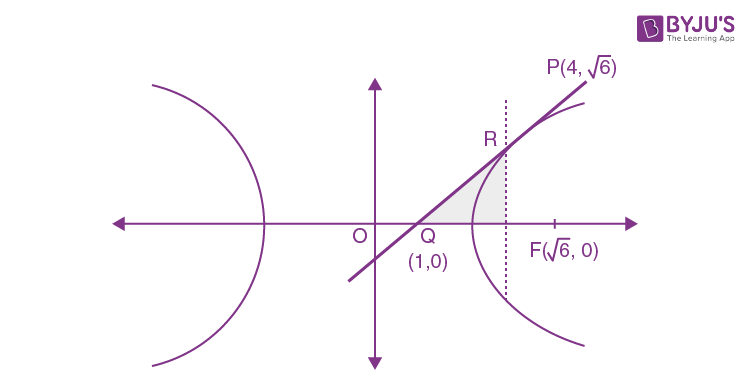Tangent at P (4, √6)

4 (x) – 2 . √6y = 4

⇒ 2x – √6y = 2 …(1)

For Q, put y = 0

Q (1,0)

Equation of Latus rectum:

X = ae = 2 (√3 / 2) = √6 …(2)

Solving (1) & (2), we get R (√6, 2 – (2 / √6))

Area of ΔQFR = (1 / 2) (√6 – 1) (2 – (2 / √6))

= (7 / √6) – 2

Question 18: Let f : R → R be a function defined as

$$\begin{array}{l}f(x)=\left\{\begin{matrix} [sin(a+1)x+sin2x]/2x, &x<0 & \\ b,& x=0 & \\ \frac{\sqrt{x+bx^{3}-\sqrt{x}}}{bx^{5/2}},&x>0 & \end{matrix}\right.\end{array}$$
. If f is continuous at x = 0, then the value of a + b is equal to

a. – 2
b. – 2 / 5
c. – 3 / 2
d. – 3

‘f’ is continuous at x = 0

⇒ f (0) = f (0) = f (0+)

f (0) = limx→0- [sin (a + 1) x + sin 2x] / 2x

limx→0- [sin (a + 1) x] / [(a + 1) x] * (a + 1) / 2 + (sin 2x / 2x)

= [(a + 1) / 2] + 1 …(1)

f (0+) = limx→0- [√(x + bx3) – √x] / bx5/2

= limx→0+ [bx3] / [bx5/2 (√(x + bx3) + √x)]

= limx→0+ [1 / (√(1 + bx2) + 1)]

= 1 / 2 …(2)

f(0) = b …(3)

From (1), (2) and (3)

[(a + 1) / 2] + 1 = 1 / 2 = b

⇒ a = -2 & b = ½

Thus, a + b = -3/2

Question 19: Let y = y (x) be the solution of the differential equation dy / dx = (y + 1) [(y + 1) ex^2/2 – x], 0 < x < 2.1, with y (2) = 0. Then the value of dy / dx at x = 1 is equal to:

a. [e5/2] / [1 + e2]2
b. [5e1/2] / [e2 + 1]2
c. – 2e2 / (1 + e2)2
d. [-e3/2] / [e2 + 1]2

dy / dx = (y + 1) [(y + 1) ex^2/2 – x]

⇒ [- 1 / (y + 1)2] (dy / dx) – x [1 / (y + 1)] = – ex^2/2

Put, 1 / (y + 1) = z

[- 1 / (y + 1)2] [dy / dx] = dz / dx

[dz / dx] + z (- x) = – ex^2/2

IF = e∫-x dx = e-x^2/2

z (e-x^2/2) = – ∫ e-x^2/2 . ex^2/2 dx = – ∫ 1 dx = – x + c

⇒ (ex^2/2 / (y + 1)) = – x + c …(1)

Given y = 0 at x = 2

Put in (1)

e-2 / (0 + 1) = – 2 + c

C = e-2 + 2 …(2)

From (1) and (2)

y + 1 = e-x^2/2 / (e-2 + 2 – x)

Again, at x = 1

y + 1 = e3/2 / [e2 + 1] [y + 1] = e3/2 / [e2 + 1]

dy / dx|x=1 = {e3/2 / [e2 + 1]} {[e3/2 / (e2 + 1)] * [e1/2 – 1]}

= – [e3/2] / [e2 + 1]2

Question 20: Let a tangent be drawn to the ellipse (x2 / 27) + y2 = 1 at (3√3 cos θ, sin θ) where θ ∈ (0, π / 2). Then the value of θ such that the sum of intercepts on axes made by a tangent is minimum is equal to:

a. π / 8
b. π / 6
c. π / 3
d. π / 4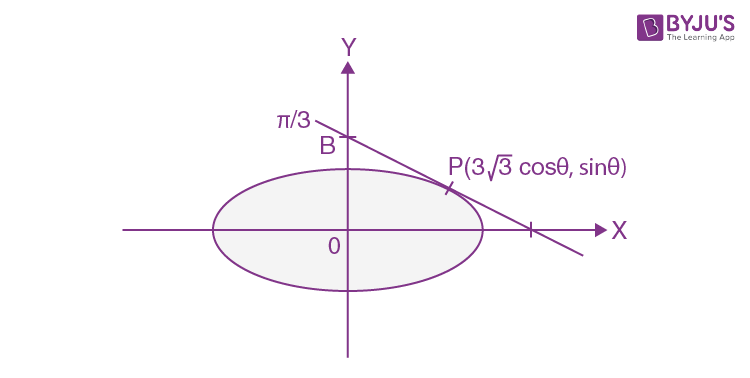Equation of tangent [x / 3√3] cos θ + y sin θ = 1

A [(3√3 / cos θ), 0], B [(0, 1 / sin θ)]

Now sum of intercept = [3√3 / cos θ] + [1 / sin θ]

Let y = 3√3 sec θ + cosec θ

y’ = 3√3 sec θ tan θ – cosec θ cot θ

y’ = 0

tan θ = 1 / √3

⇒ θ = π / 6

SECTION – B

Question 21: Let P be a plane containing the line [x – 1] / 3 = [y + 6] / 4 = [z + 5] / 2 and parallel to the line [x – 3] / 4 = [y – 2] / – 3 = [z + 5] / 7. If the point (1, -1, α) lies on the plane P, then the value of |5α| is equal to __________ .

DR’s of normal

$$\begin{array}{l}\\\vec{n}\equiv \vec{b_{1}}\times\vec{b_{2}}\\ \vec{n}=\begin{vmatrix} i &j &k \\ 3 &4 &2 \\ 4&-3 &7 \end{vmatrix}\end{array}$$

(34, -13, -25)

P ≡ 34 (x – 1) – 13 (y + 6) – 25 (z + 5)

Q (1, -1, α) lies on P.

⇒ 3 (1 – 1) – 13 (- 1 + 6) – 25 (α + 5) = 0

⇒ – 25 (α + 5) = 65

⇒ +5α = – 38

⇒ |5α| = 38

Question 22: ∑r=110 r! (r3 + 6r2 + 2r + 5) = α (11!). Then the value of α is equal to _______ .

Tr = r! ((r + 1) (r + 2) (r + 3) – 9r – 1)

= (r + 3) ! – 9r . r! – r!

= (r + 3) ! – 9 (r – 1 + 1)) r! – r!

= (r + 3) ! – 9 (r + 1) ! + 8r!

= {(r + 3) ! – (r + 1) ! – 8 (r + 1) ! – r !

Now,

r=110 Tr = {13! + 12! – 3! – 2!} – 8 {11! – 1!}

= 13! + 12! − 8 (11!)

= (13 * 12 + 12 – 8) * 11!

= 160 * 11!

α = 160

Question 23: The term independent of x in the expansion of [(x + 1) / (x2/3 – x1/3 + 1) – (x – 1) / (x – x1/2)]10, x ≠ 1, is equal to ________.

[(x1/3 + 1) – [√x + 1] / [√x]]10 = (x1/3 – x)10

General term, Tr+1 = 10Cr (x1/3)10-r (- x)r

For term independent of x

[10 – r] / 3 – (r / 2) = 0

20 – 2r – 3r = 0

r = 4

Therefore required term, T5 = 10C4 = [10 * 9 * 8 * 7] / [4 * 3 * 2 * 1] = 210

Question 24: Let nCr denote the binomial coefficient of xr in the expansion of (1 + x)n. If ∑k=010 [22 + 3k] nCk = ɑ . 310 + β . 210 then ɑ + β is equal to _______.

n must be equal to 10

k=010 [22 + 3k] nCk

= ∑k=010 [4 + 3k] nCk

= 4 ∑k=010nCk + 3 ∑k=010 k nCk

= 4 (210) + 3 * 10 * 29

= 19 × 210

∴ α = 0 and β = 19

Thus, α + β = 19

Question 25: Let P (x) be a real polynomial of degree 3 which vanishes at x = – 3. Let P(x) have local minima at x = 1, local maxima at x = -1 and ∫-11 P (x) dx = 18, then the sum of all the coefficients of the polynomial P (x) is equal to _______________ .

P’ (x) = a (x + 1) (x – 1)

P (x) = (ax3 / 3) – ax + c

P (-3) = 0 (given)

⇒ a(- 9 + 3) + C = 0

⇒ 6a = C …(i)

Also, ∫-11 P (x) dx = 18 ⇒ ∫-11 [a ((x3 / 3) – x) + c] dx = 18

⇒ 0 + 2 C = 18 ⇒ C = 9

From (i), a = 3 / 2

P (x) = (x3 / 2) – (3 / 2) x + 9

Sum of co-efficient = – 1 + 9 = 8

Question 26: Let the mirror image of the point (1, 3, a) with respect to the plane r . (2i – j + k) – b = 0 be (-3, 5, 2). Then, the value of |a + b| is equal to ______.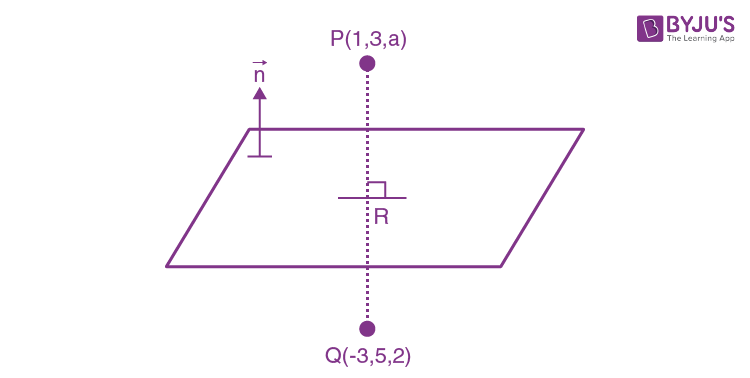Plane : 2x -y + z = b

R ≡ [- 1, 4, (a + 2) / 2] on plane

– 2 – 4 + (a + 2) / 2 = b

⇒ a + 2 = 2b + 12 ⇒ a = 2b + 10 …(1)

PQ < 4, -2, a -2>

(2 / 4) = – 1 / – 2 = 1 / (a – 2)

a- 2 = 2

a = 4, b = – 3

|a + b| = 1

Question 27: If f (x) and g (x) are two polynomials such that the polynomial P (x) = f (x3) + x g (x3) is divisible by x2 + x + 1, then P (1) is equal to _______.

Roots of x2 + x + 1 are ω and ω2 now

Q (ω) = f (1) + ω g (1) = 0 …(1)

Q (ω2) = f (1) + ω2 g (1) = 0 …(2)

2 f (1) – g (1) = 0

g (1) = 2 f (1)

f (1) = g (1) = 0

Therefore , Q (1) = f (1) + g (1)

= 0 + 0

= 0

Question 28: Let I be an identity matrix of order 2 × 2 and

$$\begin{array}{l}P=\begin{bmatrix} 2 &-1 \\ 5&-3 \end{bmatrix}\end{array}$$
. Then the value of n ∈ N for which Pn = 5I – 8P is equal to ________.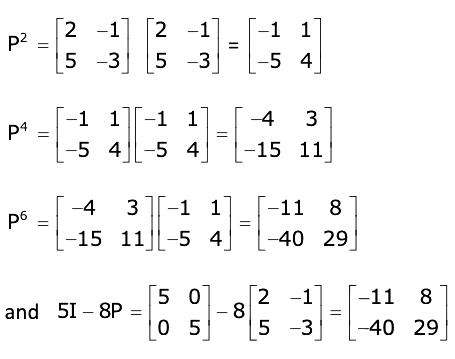P6 = 5I – 8P

Thus, n = 6

Question 29: Let f : R → R satisfy the equation f (x + y) = f (x) . f (y) for all x, y ∈ R and f (x) ≠ 0 for any x ∈ R. If the function f is differentiable at x = 0 and f’ (0) = 3, then limh→0 (1 / h) [f (h) – 1] is equal to _______.

f (x + y) = f (x) . f (y) then

f (x) = akx

f’(x) = (akx ) k ln a

f’(0) = k ln a = 3 (given f’(0) = 3)

a = e3/k

f (x) = (e3/k)kx = e3x

Now, limh→0 [f (h) – 1] / h = limh→0 {[e3h – 1] / 3h} * 3 = 1 * 3 = 3

Question 30: Let y = y (x) be the solution of the differential equation xdy – ydx = √(x2 – y2) dx, x ≥ 1, with y (1) = 0. If the area bounded by the line x = 1, x = eπ, y = 0 and y = y (x) is αe + β, then the value of 10 (α + β) is equal to ________.

xdy – ydx = √(x2 – y2) dx

[xdy – ydx] / x2 = (1 / x) √1 – (y2 / x2) dx [∫ d (y / x) / √1 – (y2 / x2) = ∫ dx / x]

⇒ sin-1 (y / x) = ln |x| + c

At x = 1, y = 0 ⇒ c = 0

y = x sin (l nx)

A = ∫1e^π x sin (ln x) dx

x = et, dx = et dt = ∫0π e2t sin (t) dt

αe + β = [(e2t / 5) [2 sin t – cos t])0π = [1 + e] / 5

α = 1 / 5, β = 1 / 5

Thus, 10 (α + β) = 4